# Digital Image Processing Basics

## 1 基本概念

《数字图像处理（第三版），冈萨雷斯》
Image Processing, Analysis, and Machine Vision, 3rd Edition, 2007

### 1.1 什么是数字图像、像素

$f(x,y) = \left( \begin{array}{cccc} f(0,0) & f(0,1) & \cdots & f(0,N-1) \\ f(1,0) & f(1,1) & \cdots & f(1,N-1) \\ \vdots & \vdots & \ddots & \vdots \\ f(M-1,0) & f(M-1,1) & \cdots & f(M-1,N-1) \end{array} \right)$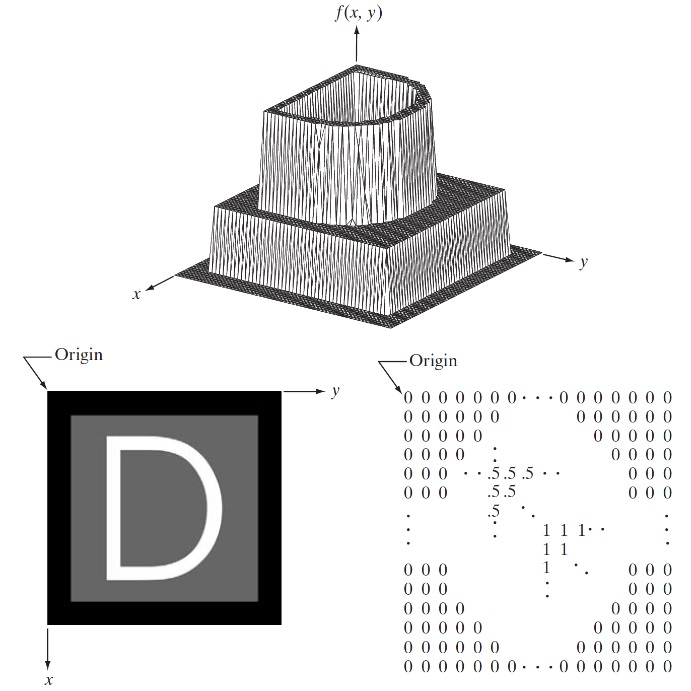Figure 1: 图像的表示（矩阵中0/0.5/1分别表示黑色/灰色/白色）

### 1.2 像素间的一些基本关系

#### 1.2.1 距离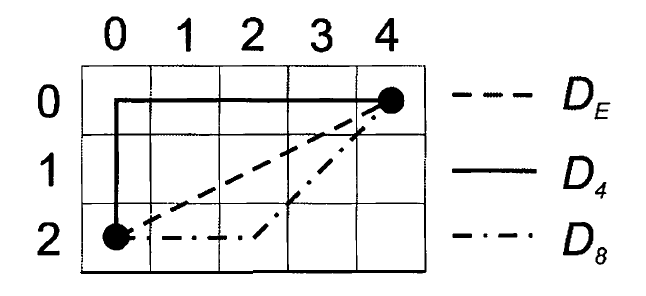Figure 2: 欧氏距离、城区距离、棋盘距离的实例

### 1.4 图像插值(Image Interpolation)

#### 1.4.2 双线性插值(Bilinear interpolation)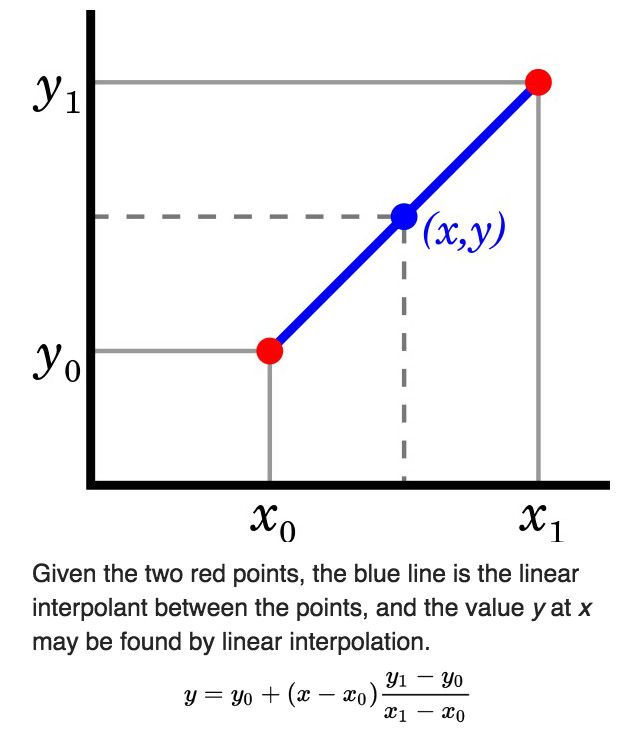Figure 3: 线性插值实例

\begin{aligned} I_{(20, 14.5)} = \frac{15-14.5}{15-14} \cdot 91 + \frac{14.5 - 14}{15 -14} \cdot 210 = 150.5 \\ I_{(21, 14.5)} = \frac{15-14.5}{15-14} \cdot 162 + \frac{14.5-14}{15-14} \cdot 95 = 128.5 \end{aligned}

$I_{(20.2, 14.5)} = \frac{21-20.2}{21-20} \cdot 150.5 + \frac{20.2-20}{21-20} \cdot 128.5 = 146.1$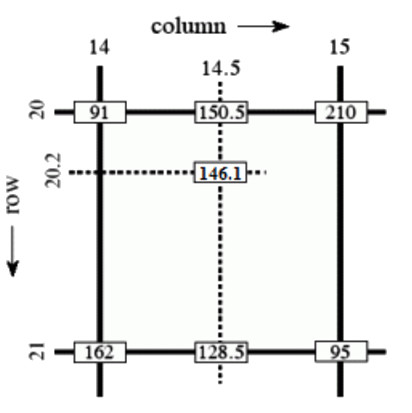Figure 4: 双线性插值实例

## 2 灰度变换和空间滤波(Intensity Transformation and Spatial Filtering)

### 2.1 基本的灰度变换函数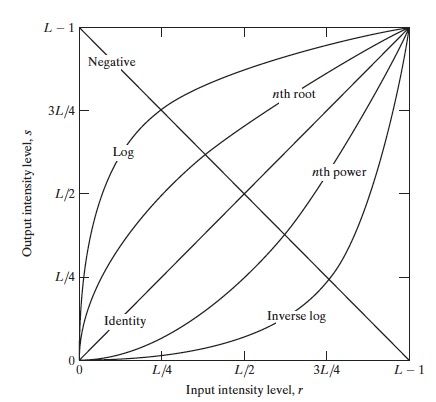Figure 5: 基本的灰度变换函数

#### 2.1.1 反转变换

$s = L-1-r$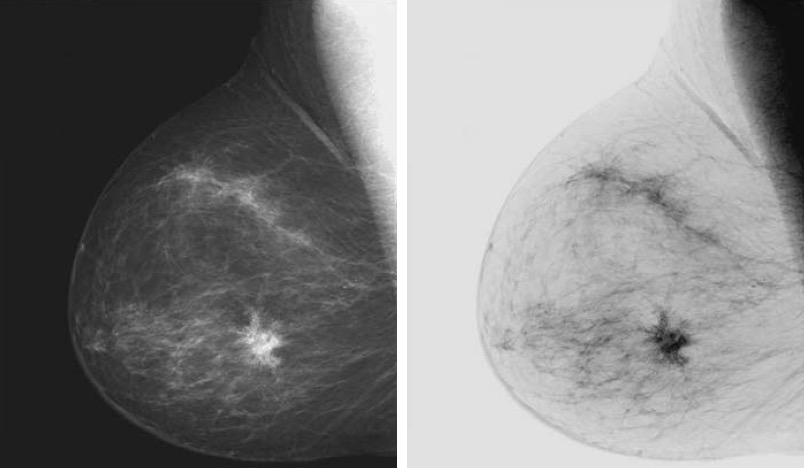Figure 6: 乳房X线照片及其反转图像（其反转图像更容易分析）

#### 2.1.2 对数变换

$s = c \log(1+r)$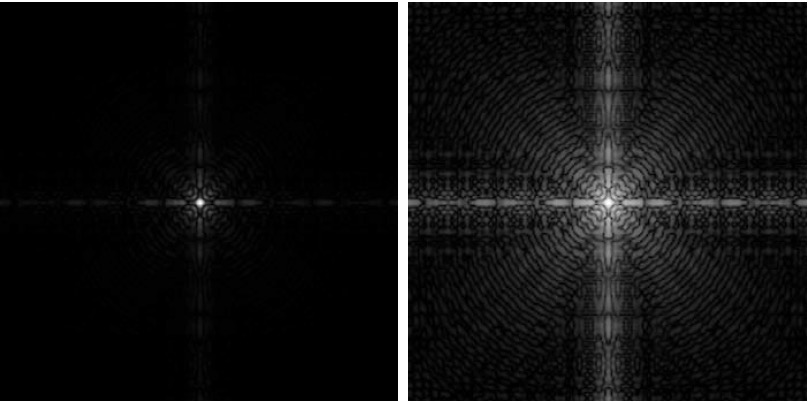Figure 7: 傅里叶频谱（左图），及应用对数变换(c=1)后的结果（右图）

#### 2.1.3 幂律（伽马）变换

$s = c r^{\gamma}$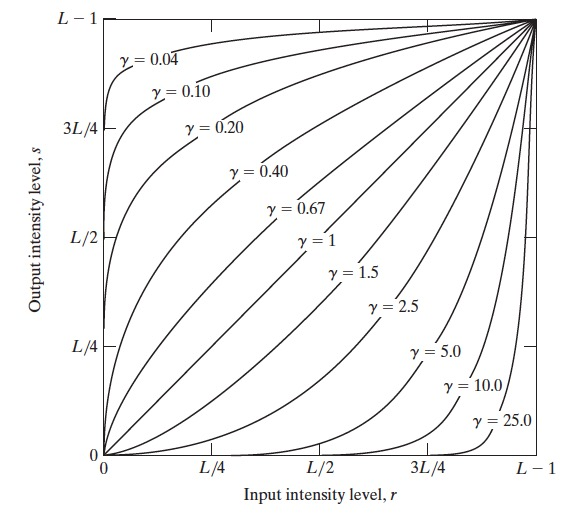Figure 8: 不同伽马 $$\gamma$$ 值（假设所有情形c=1）的 $$s = c r^{\gamma}$$ 曲线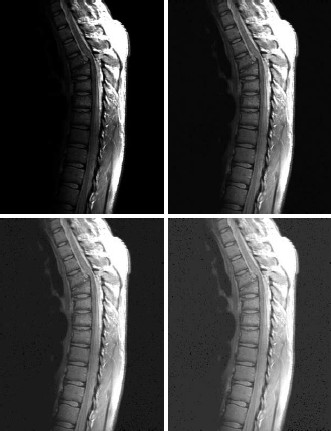Figure 9: 伽马变换实例（增加暗部对比度）：左上角为原图，其它3个是伽马变换后的图像（c均为1， $$\gamma$$ 值分别为0.6,0.4,0.3）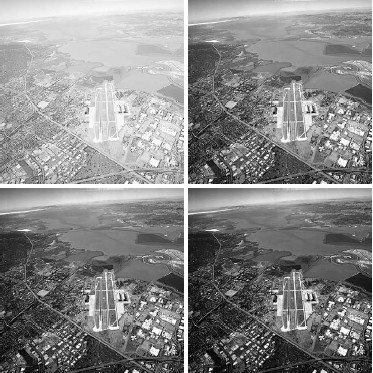Figure 10: 伽马变换实例（增加亮部对比度）：左上角为原图，其它3个是伽马变换后的图像（c均为1， $$\gamma$$ 值分别为3.0,4.0,5.0）

### 2.2 直方图处理(Histogram Processing)

$p(r_k) = \frac{n_k}{MN}$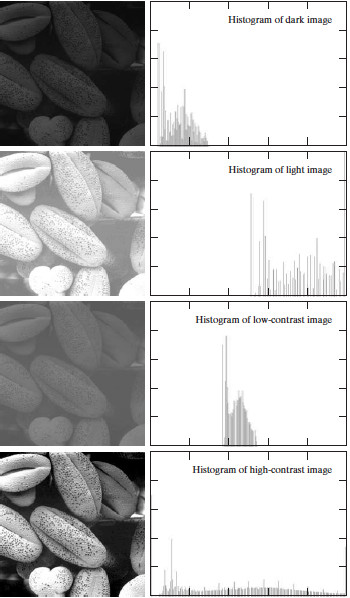Figure 11: 图像及其直方图（横轴为灰度值，竖轴为对应像素的个数）

#### 2.2.1 直方图均衡(Histogram Equalization)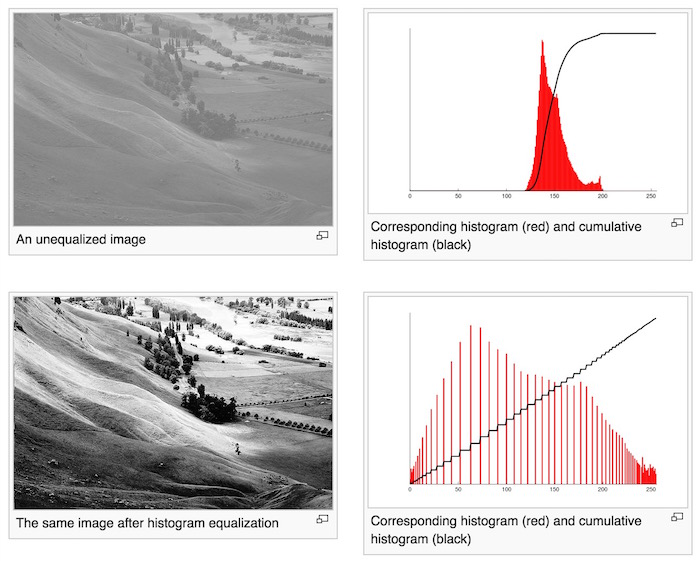Figure 12: 直方图均衡实例（处理后图像的直方图累积分布函数基本上是一条“直线”，实例摘自Wikipedia

13 是直方图均衡的另一个例子。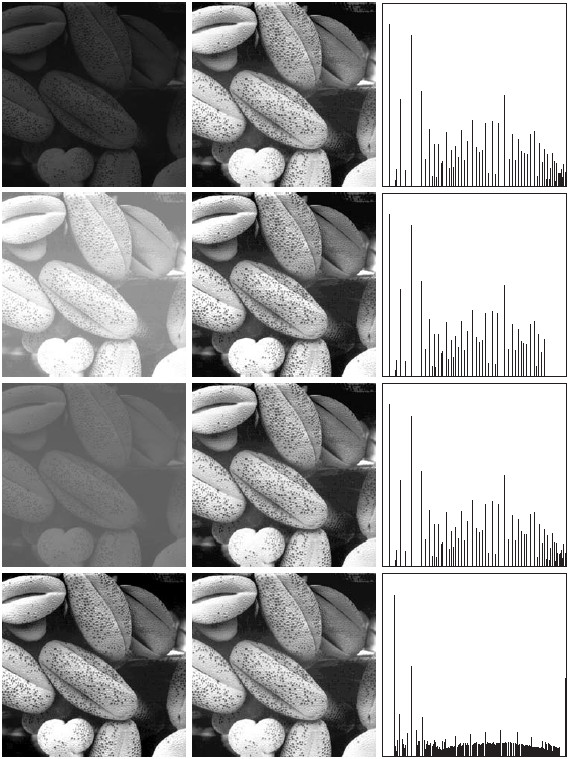Figure 13: 直方图均衡实例（左边是原图，中间是均衡后的图像，右边是中间图像的直方图）

##### 2.2.1.1 直方图均衡算法

\begin{equation} s_k = T(r_k) = (L-1) \sum_{j=0}^{k}\frac{n_j}{MN}, \; k = 0,1,2,\cdots,L-1 \tag{Histogram Equalization Formula} \end{equation}

##### 2.2.1.2 直方图均衡算法实例

Figure 14: 直方图均衡实例，原图的灰度矩阵

52 1 1/64 1/64 255*(1/64)=3.98 4
55 3 3/64 4/64 255*(4/64)=15.93 16
58 2 2/64 6/64 255*(6/64)=23.90 24
59 3 3/64 9/64 255*(9/64)=35.85 36
60 1 1/64 10/64 255*(10/64)=39.84 40
61 4 4/64 14/64 255*(14/64)=55.78 56
62 1 1/64 15/64 255*(15/64)=59.76 60
63 2 2/64 17/64 255*(17/64)=67.73 68
64 2 2/64 19/64 255*(19/64)=75.70 76
65 3 3/64 22/64 255*(22/64)=87.65 88
66 2 2/64 24/64 255*(24/64)=95.62 96
67 1 1/64 25/64 255*(25/64)=99.60 100
68 5 5/64 30/64 255*(30/64)=119.53 120
69 3 3/64 33/64 255*(33/64)=131.48 131
70 4 4/64 37/64 255*(37/64)=147.42 147
71 2 2/64 39/64 255*(39/64)=155.39 155
72 1 1/64 40/64 255*(40/64)=159.37 159
73 2 2/64 42/64 255*(42/64)=167.34 167
75 1 1/64 43/64 255*(43/64)=171.32 171
76 1 1/64 44/64 255*(44/64)=175.31 175
77 1 1/64 45/64 255*(45/64)=179.29 179
78 1 1/64 46/64 255*(46/64)=183.28 183
79 2 2/64 48/64 255*(48/64)=191.25 191
83 1 1/64 49/64 255*(49/64)=195.23 195
85 2 2/64 51/64 255*(51/64)=203.20 203
87 1 1/64 52/64 255*(52/64)=207.18 207
88 1 1/64 53/64 255*(53/64)=211.17 211
90 1 1/64 54/64 255*(54/64)=215.15 215
94 1 1/64 55/64 255*(55/64)=219.14 219
104 2 2/64 57/64 255*(57/64)=227.10 227
106 1 1/64 58/64 255*(58/64)=231.09 231
109 1 1/64 59/64 255*(59/64)=235.07 235
113 1 1/64 60/64 255*(60/64)=239.06 239
122 1 1/64 61/64 255*(61/64)=243.04 243
126 1 1/64 62/64 255*(62/64)=247.03 247
144 1 1/64 63/64 255*(63/64)=251.01 251
154 1 1/64 64/64 255*(64/64)=255 255

##### 2.2.1.3 直方图均衡优缺点

1）变换后图像的灰度级可能减少，某些细节可能丢失；
2）它对处理的数据不加选择，它可能会增加背景噪声的对比度并且降低有用信号的对比度；
3）某些图像，如直方图有高峰，经处理后对比度不自然的过分地增强了。

#### 2.2.2 Histogram Matching (Specification)

15 是一个“直方图均衡”处理后效果不是很好的例子。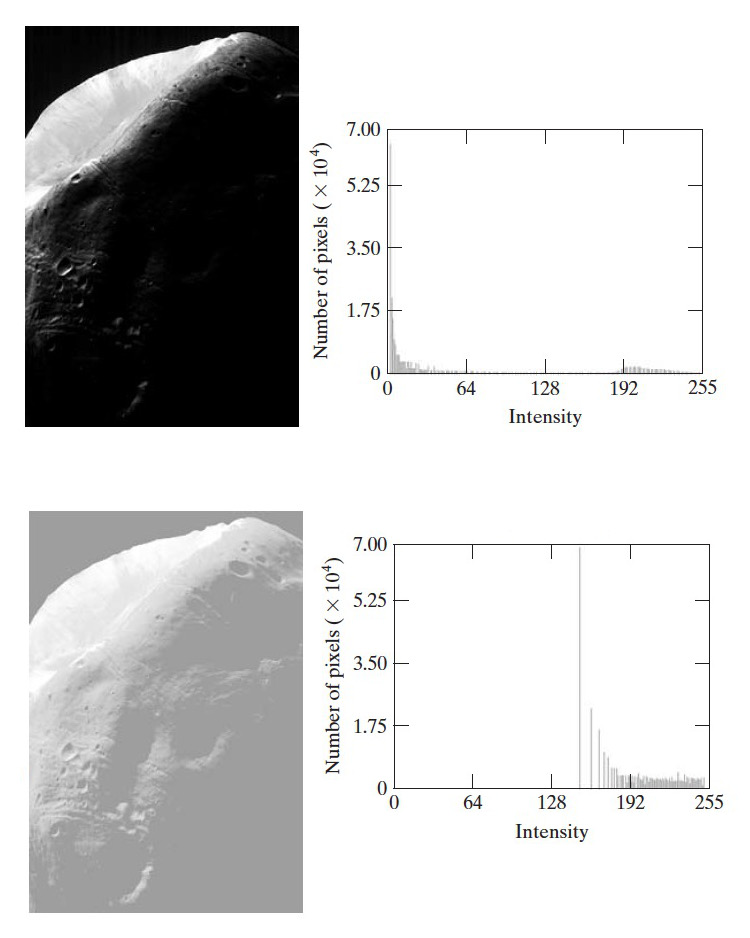Figure 15: 一幅很黑的图像（上图）在“直方图均衡”处理后（下图）变得灰蒙蒙

#### 2.2.3 直方图统计（灰度均值，灰度方差）

$m = \sum_{i=0}^{L-1} r_i p(r_i)$

$\sigma^2 = \sum_{i=0}^{L-1} (r_i - m)^2 p(r_i)$

$m = \frac{1}{MN} \sum_{x=0}^{M-1} \sum_{y=0}^{N-1} f(x,y)$

$\sigma^2 = \frac{1}{MN} \sum_{x=0}^{M-1} \sum_{y=0}^{N-1} [ f(x,y) - m ]^2$

### 2.3 空间滤波基础

$g(x,y) = T[f(x,y)]$

#### 2.3.1 空间滤波机理

(1)一个邻域（典型地是一个较小的矩形）；
(2)对该邻域包围的图像像素执行的预定义操作。

##### 2.3.1.1 线性空间滤波器（其操作可概括为“乘积求和”）

16 说明了使用 $$3 \times 3$$ 邻域的线性空间滤波器的机理。在图像中的任意一点 $$(x,y)$$ ，滤波器的响应 $$g(x,y)$$ 是滤波器系数与由该滤波器包围的图像像素的乘积之和：
$g(x,y) = w(-1,-1)f(x-1,y-1) + w(-1,0)f(x-1,y) + \cdots + w(0,0)f(x,y) + \cdots + w(1,1) f(x+1,y+1)$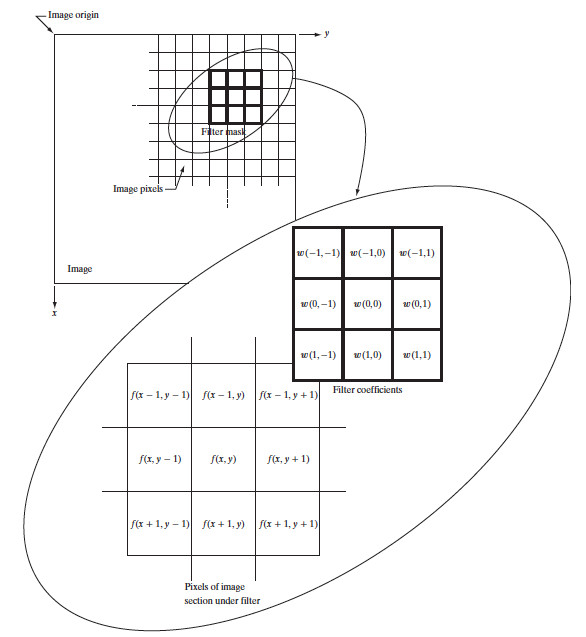Figure 16: 使用大小为 $$3 \times 3$$ 的滤波器模板的线性空间滤波器

$g(x,y) = \sum_{s=-a}^{a} \sum_{t=-b}^{b} w(s,t) f(x+s, y+t)$

#### 2.3.2 线性空间滤波的相关与卷积(Spatial Correlation and Convolution)

\begin{equation} w(x,y) \mathbin{☆} f(x,y) = \sum_{s=-a}^{a} \sum_{t=-b}^{b} w(s,t) f(x+s, y+t) \tag{Correlation Formula} \end{equation}

\begin{equation} w(x,y) \star f(x,y) = \sum_{s=-a}^{a} \sum_{t=-b}^{b} w(s,t) f(x-s, y-t) \tag{Convolution Formula} \end{equation}

##### 2.3.2.1 “相关”和“卷积”实例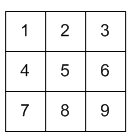Figure 17: Input f(x,y)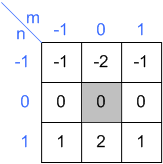Figure 18: Kernel w(x,y)

“相关”操作 $$w(x,y) \mathbin{☆} f(x,y)$$ 的计算过程如图 19 所示。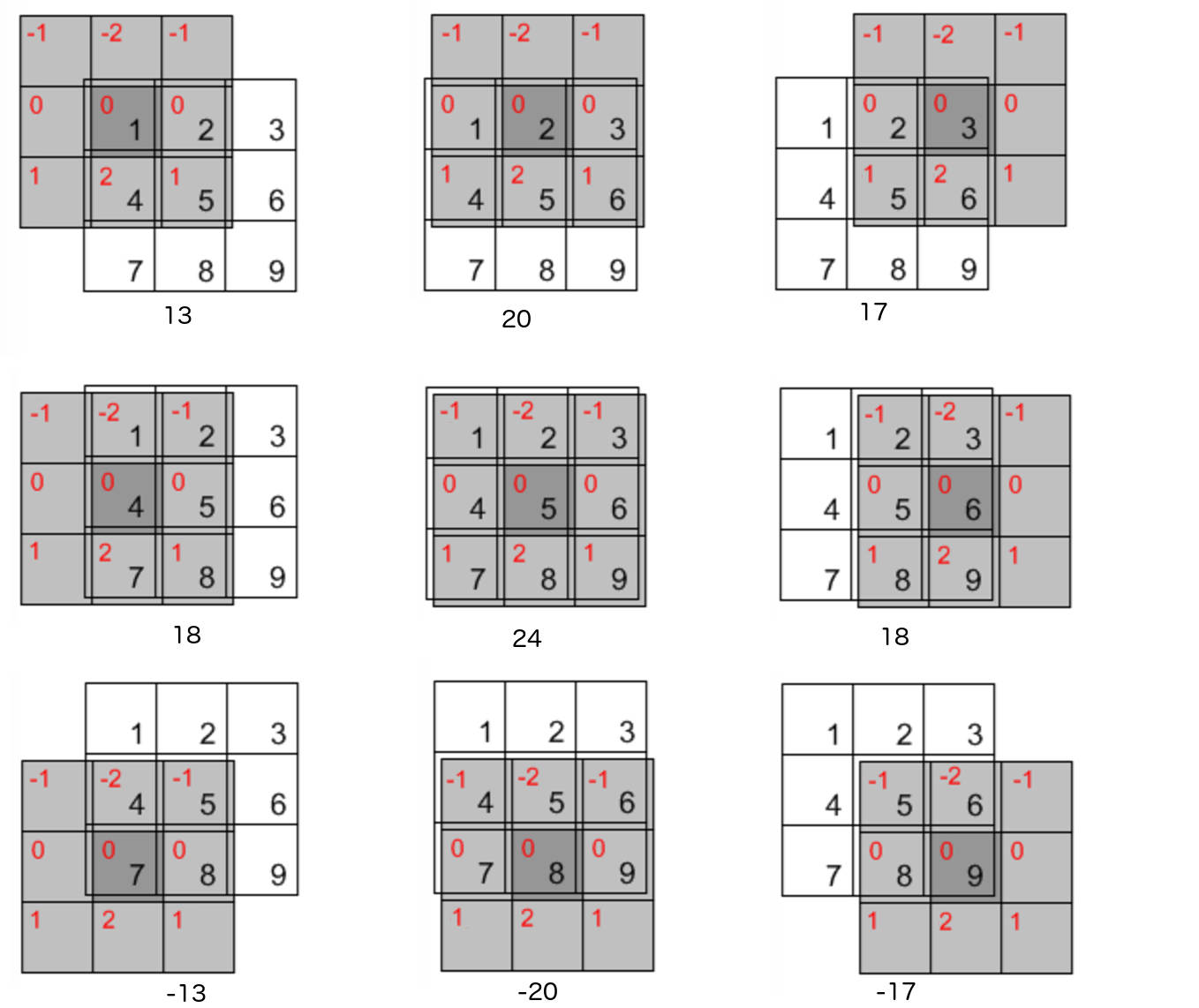Figure 19: “相关”操作 $$w(x,y) \mathbin{☆} f(x,y)$$

\begin{aligned}g_1(0,0) &= 0 \times (-1) + 0 \times (-2) + 0 \times (-1) + 0 \times 0 + 1 \times 0 + 2 \times 0 + 0 \times 1 + 4 \times 2 + 5 \times 1 = 13 \\ g_1(0,1) &= 0 \times (-1) + 0 \times (-2) + 0 \times (-1) + 1 \times 0 + 2 \times 0 + 3 \times 0 + 4 \times 1 + 5 \times 2 + 6 \times 1 = 20 \\ g_1(0,2) &= 0 \times (-1) + 0 \times (-2) + 0 \times (-1) + 2 \times 0 + 3 \times 0 + 0 \times 0 + 5 \times 1 + 6 \times 2 + 0 \times 1 = 17 \\ g_1(1,0) &= 0 \times (-1) + 1 \times (-2) + 2 \times (-1) + 0 \times 0 + 4 \times 0 + 5 \times 0 + 0 \times 1 + 7 \times 2 + 8 \times 1 = 18 \\ g_1(1,1) &= 1 \times (-1) + 2 \times (-2) + 3 \times (-1) + 4 \times 0 + 5 \times 0 + 6 \times 0 + 7 \times 1 + 8 \times 2 + 9 \times 1 = 24 \\ g_1(1,2) &= 2 \times (-1) + 3 \times (-2) + 0 \times (-1) + 5 \times 0 + 6 \times 0 + 0 \times 0 + 8 \times 1 + 9 \times 2 + 0 \times 1 = 18 \\ g_1(2,0) &= 0 \times (-1) + 4 \times (-2) + 5 \times (-1) + 0 \times 0 + 7 \times 0 + 8 \times 0 + 0 \times 1 + 0 \times 2 + 0 \times 1 = -13 \\ g_1(2,1) &= 4 \times (-1) + 5 \times (-2) + 6 \times (-1) + 7 \times 0 + 8 \times 0 + 9 \times 0 + 0 \times 1 + 0 \times 2 + 0 \times 1 = -20 \\ g_1(2,2) &= 5 \times (-1) + 6 \times (-2) + 0 \times (-1) + 8 \times 0 + 9 \times 0 + 0 \times 0 + 0 \times 1 + 0 \times 2 + 0 \times 1 = -17 \end{aligned}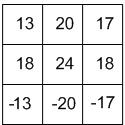Figure 20: Correlation Output

“卷积”操作和“相关”操作是类似的，唯一不同在于做“卷积”操作时要提前把滤波器旋转（包括水平方向和垂直方向） $$180^{\circ}$$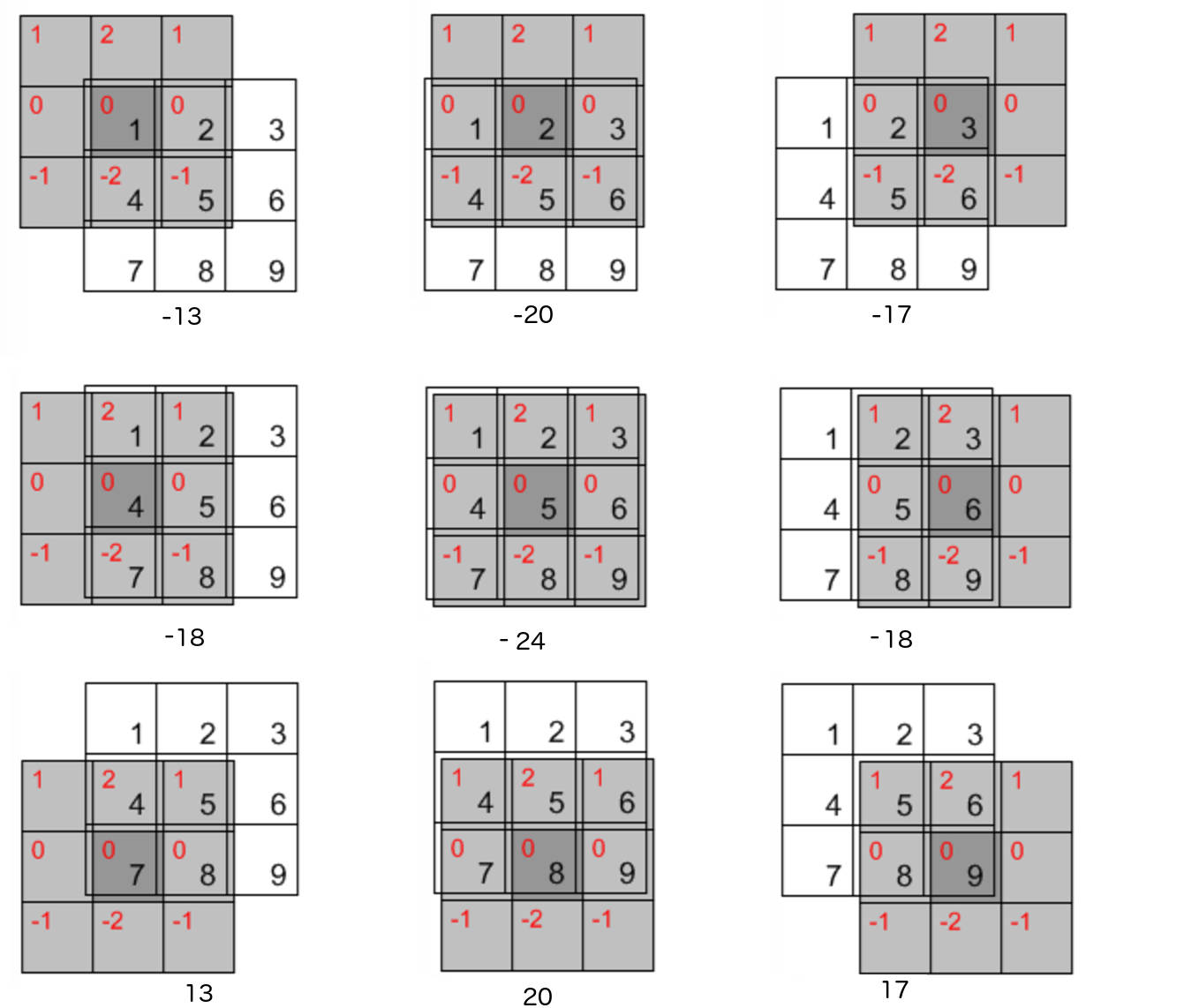Figure 21: “卷积”操作 $$w(x,y) \star f(x,y)$$ （滤波器已经旋转了 $$180^{\circ}$$ ）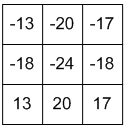Figure 22: Convolution Output

#### 2.3.3 应用软件对线性空间滤波的支持

ImageMagick和GIMP支持定制线性空间滤波的模板。

### 2.4 平滑空间滤波器

#### 2.4.1 平滑线性滤波器（均值滤波器）

23 是两个 $$3 \times 3$$ 平滑线性滤波器的示例。左边的平滑线性滤波器的所有系数都相等（都为 $$1/9$$ ），有时也称为盒状滤波器(Box Filter)。右边的平滑线性滤波器实现是的“加权平均”，突出了处于模板中心位置的像素的重要性比其它像素的重要性要更大。不过，实践中，由于模板在一幅图像中任何一个位置所跨过的区域很小，通常很难看出使用图 23 中两个模板或类似方式进行平滑处理后的图像的区别。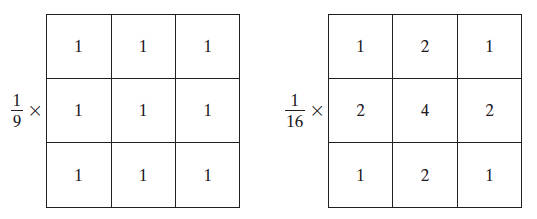Figure 23: 两个 $$3 \times 3$$ 平滑线性滤波器的示例

24 是使用不同尺寸的模板对图像进行平滑处理后的效果。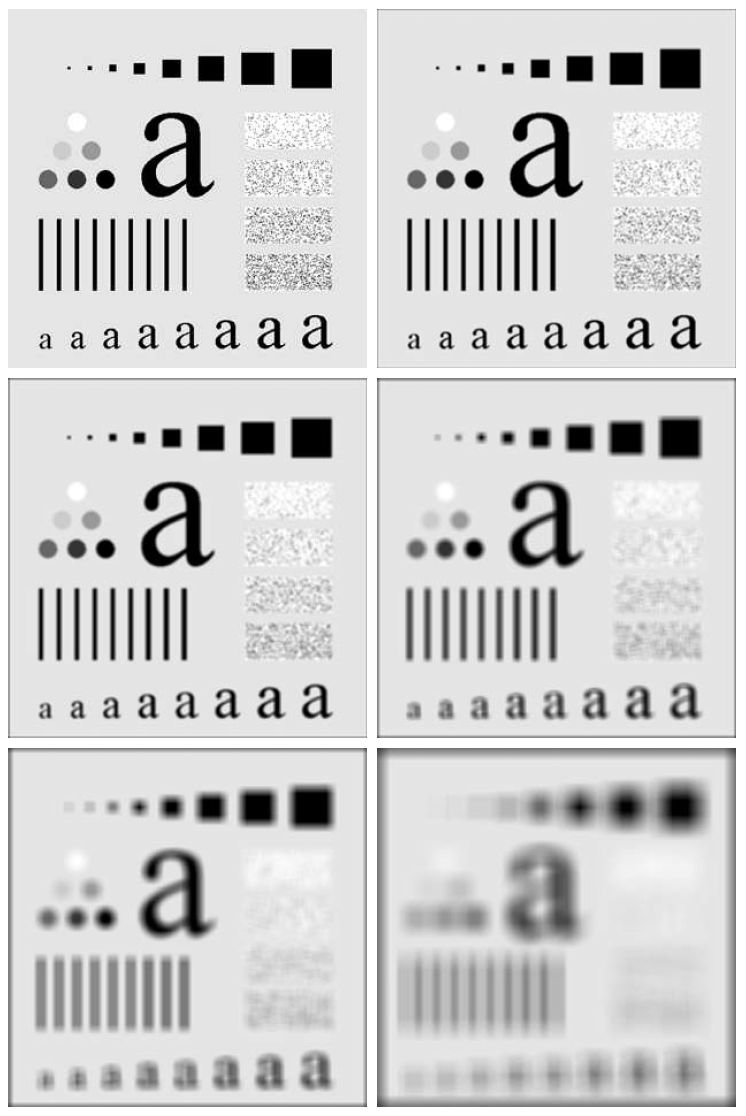Figure 24: 不同尺寸的模板对图像进行平滑处理后的效果（第一个图是原图像，其它是分别使用 m=3,5,9,15,35 的方形均值滤波器处理后的效果）

#### 2.4.2 高斯模糊(Gaussian blur)

$G(x,y) = \frac{1}{2 \pi \sigma^2} e^{- \frac{x^2 + y^2}{2 \sigma^2}}$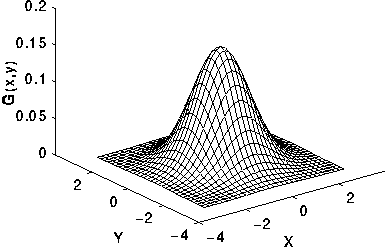Figure 25: 二维高斯函数

26 是高斯滤波器（标准差 $$\sigma=1$$ ，核大小为 $$5\times 5$$ ）的一个离散近似。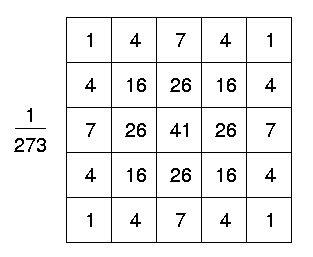Figure 26: 高斯滤波器（标准差 $$\sigma=1$$ ，核大小为 $$5\times 5$$ ）

 6.7e-07 2.292e-05 0.00019117 0.00038771 0.00019117 2.292e-05 6.7e-07 2.292e-05 0.00078634 0.00655965 0.0133037 0.00655965 0.00078633 2.292e-05 0.00019117 0.00655965 0.0547216 0.110982 0.0547216 0.00655965 0.00019117 0.00038771 0.0133037 0.110982 0.225084 0.110982 0.0133037 0.00038771 0.00019117 0.00655965 0.0547216 0.110982 0.0547216 0.00655965 0.00019117 2.292e-05 0.00078633 0.00655965 0.0133037 0.00655965 0.00078633 2.292e-05 6.7e-07 2.292e-05 0.00019117 0.00038771 0.00019117 2.292e-05 6.7e-07

#### 2.4.3 统计排序（非线性）滤波器

##### 2.4.3.1 中值滤波器（适合去除椒盐噪声）

27 是用均值滤波器和中值滤波器处理被椒盐噪声污染的图像的示例，显然用中值滤波器处理后的效果要更好。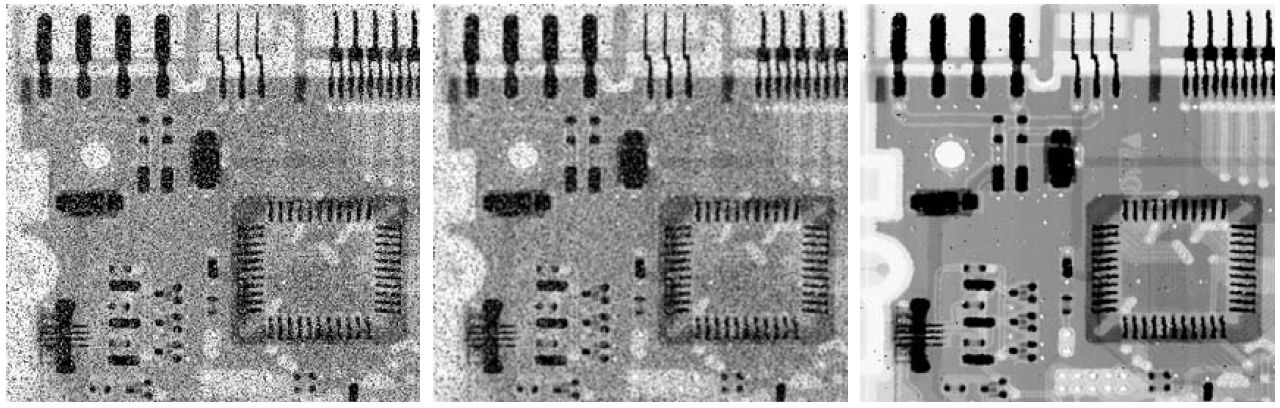Figure 27: 左图为被椒盐噪声污染的电路板，中图为用均值滤波器处理后的图像，右图为用中值滤波器处理后的图像

### 2.5 锐化空间滤波器

#### 2.5.1 数字函数微分基础

$\frac{\partial f}{\partial x} = f(x+1) - f(x)$

\begin{aligned} \frac{\partial^2 f}{\partial x^2} & = f'(x+1) - f'(x) \\ & = f(x+2) - f(x+1) - f(x+1) + f(x) \\ & = f(x+2) - 2f(x+1) + f(x) \\ \end{aligned}

$\frac{\partial^2 f}{\partial x^2} = f(x+1) + f(x-1) - 2f(x)$

28 是一幅图像中一段水平灰度剖面对应一维数字函数的一阶微分和二阶微分。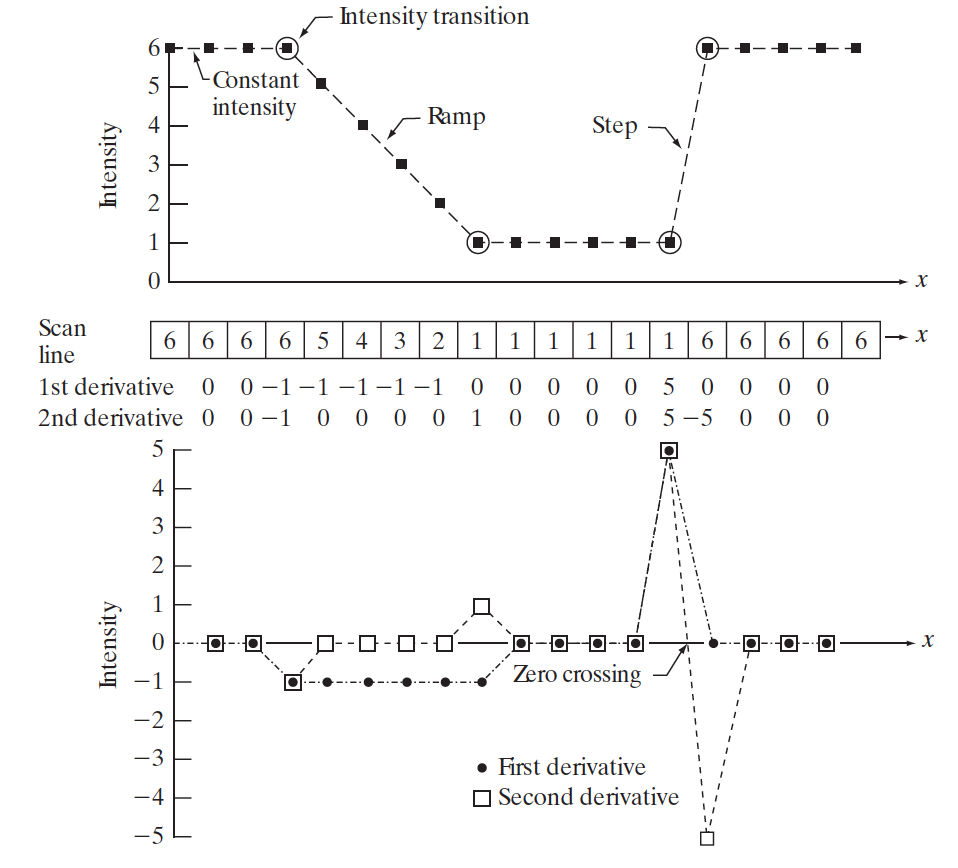Figure 28: 一幅图像中一段水平灰度剖面对应一维数字函数的一阶微分和二阶微分

#### 2.5.2 使用二阶微分进行图像锐化——拉普拉斯算子

$\nabla^2 f = \frac{\partial^2 f}{\partial x^2} + \frac{\partial^2 f}{\partial y^2}$

$\frac{\partial^2 f}{\partial x^2} = f(x+1,y) + f(x-1,y) - 2f(x,y)$

$\frac{\partial^2 f}{\partial y^2} = f(x,y+1) + f(x,y-1) - 2f(x,y)$

$\nabla^2 f(x,y) = f(x+1,y) + f(x-1,y) + f(x,y+1) + f(x,y-1) - 4f(x,y)$
29 所示模板是上面离散拉普拉斯算子的滤波模板实现。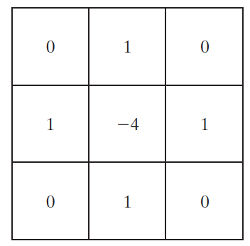Figure 29: 拉普拉斯算子的滤波模板

30 是拉普拉斯图像的一个例子。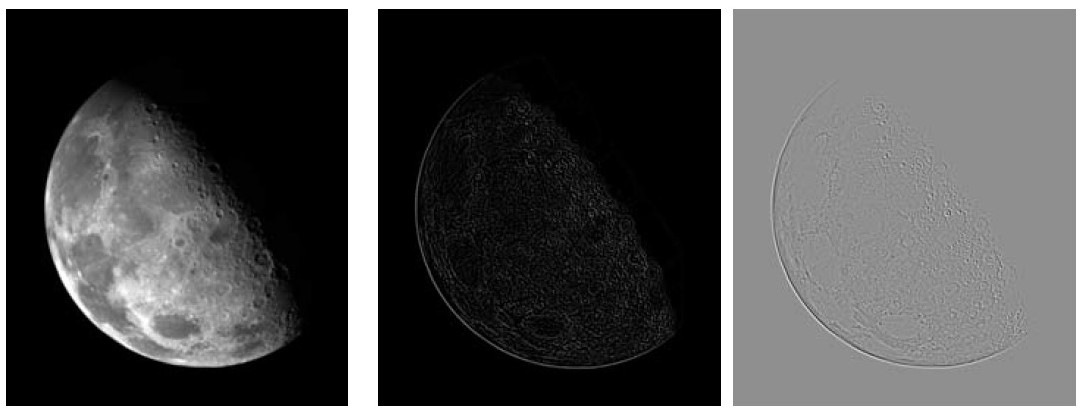Figure 30: 左图为原图，中间为拉普拉斯图像（出现负数时直接修剪为0），右边是映射到整个灰色范围[0,L-1]的拉普拉斯图像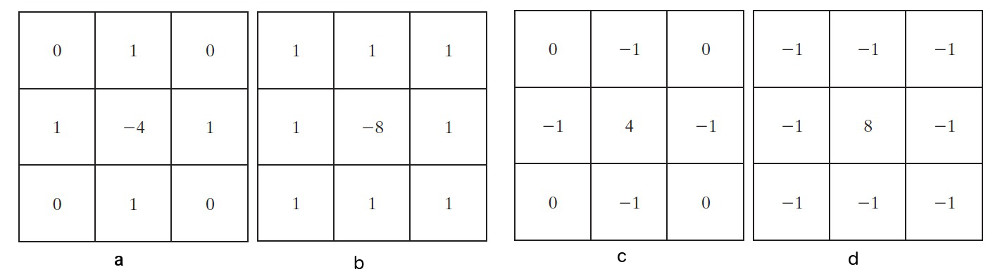Figure 31: 拉普拉斯滤波模板实现 (a)拉普拉斯“基本模板”；(b)实现带有对角项的拉普拉斯“扩展模板”；(c)和(d)是常用的其他两个拉普拉斯实现

$g(x,y) = \begin{cases} f(x,y) - \nabla^2 f(x,y) \qquad \text{如果拉普拉斯模板的中心系数为负} \\ f(x,y) + \nabla^2 f(x,y) \qquad \text{如果拉普拉斯模板的中心系数为正} \end{cases}$

32 是用拉普拉斯滤波模板进行锐化处理的实例。从图中可知，右图锐化效果更加明显，因为它使用的是拉普拉斯“扩展模板”，在对角方向上产生了额外的锐化。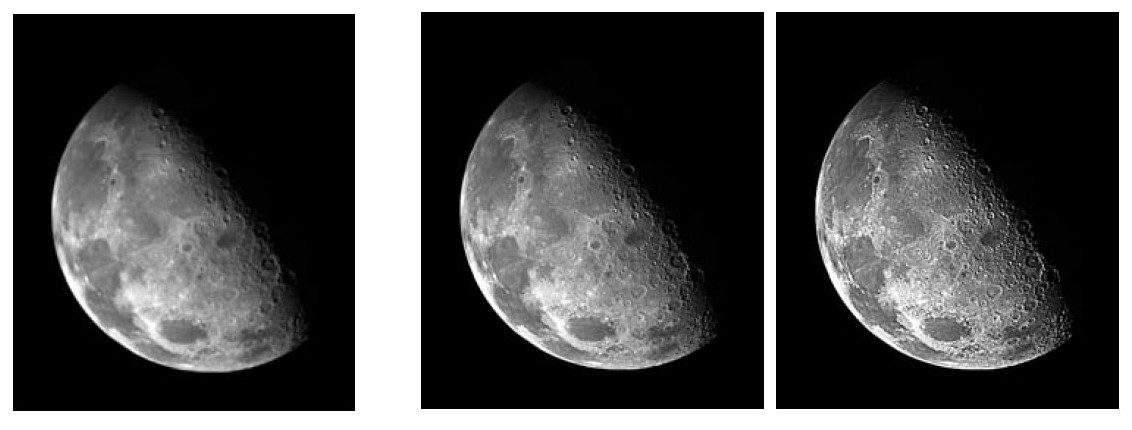Figure 32: 拉普拉斯锐化实例（左图是原图，中图是使用拉普拉斯“基本模板”锐化处理效果图，右图是拉普拉斯“扩展模板”锐化处理效果图）

##### 2.5.2.1 各向同性滤波器(Isotropic Filter)

31 中4个拉普拉斯滤波器的实现中，其中(a)和(c)对 $$90^{\circ}$$ 增幅的结果是各向同性的，(b)和(d)对 $$45^{\circ}$$ 增幅的结果是各向同性的。

#### 2.5.3 非锐化掩蔽(Unsharp Masking)和高提升滤波(Highboost Filtering)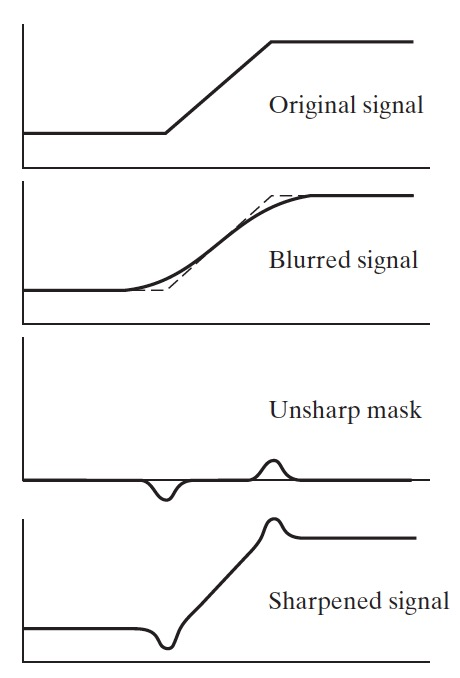Figure 33: 非锐化掩蔽的原理（将原始信号减去模糊后的信号得到钝化模板，将原信号加上钝化模板将得到锐化后的信号）

$g_{\text{mask}}(x,y) = f(x,y) - \overline{f}(x,y)$

$g(x,y) = f(x,y) + k \times g_{\text{mask}}(x,y) \qquad (k \ge 0)$

#### 2.5.4 使用一阶微分进行图像锐化（非线性）——梯度

$\nabla f \equiv \text{grad}(f) \equiv \begin{bmatrix} \frac{\partial f}{\partial x} \\ \frac{\partial f}{\partial y} \end{bmatrix}$

$M(x,y) = \sqrt{ \left(\frac{\partial f}{\partial x} \right)^2 + \left(\frac{\partial f}{\partial y} \right)^2}$

$M(x,y) \approx |\frac{\partial f}{\partial x}| + |\frac{\partial f}{\partial y}|$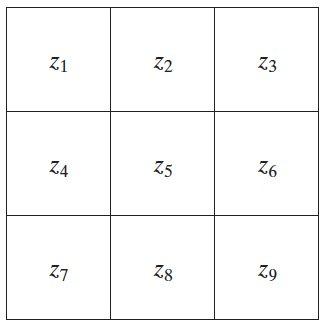Figure 34: 一幅图像的 $$3 \times 3$$ 区域，中心点 $$z_5$$ 表示任意位置 $$(x,y)$$ 处的 $$f(x,y)$$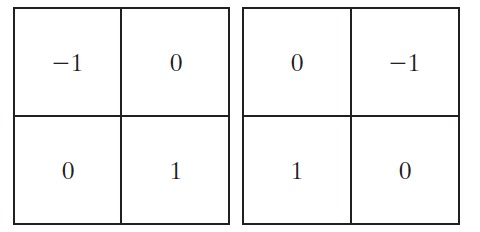Figure 35: Roberts cross gradient operators

$M(x,y) = \sqrt{(z_9 - z_5)^2 + (z_8 - z_6)^2}$

$M(x,y) = |z_9 - z_5| + |z_8 - z_6|$

##### 2.5.4.1 Sobel Operator

Sobel算子 是一个流行的梯度算子。它使用下面公式对一阶微分进行近似：
\begin{aligned} \frac{\partial f}{\partial x} &= (z_7 + 2 z_8 + z_9) - (z_1 + 2 z_2 + z_3) \\ \frac{\partial f}{\partial y} &= (z_3 + 2 z_6 + z_9) - (z_1 + 2 z_4 + z_7) \end{aligned}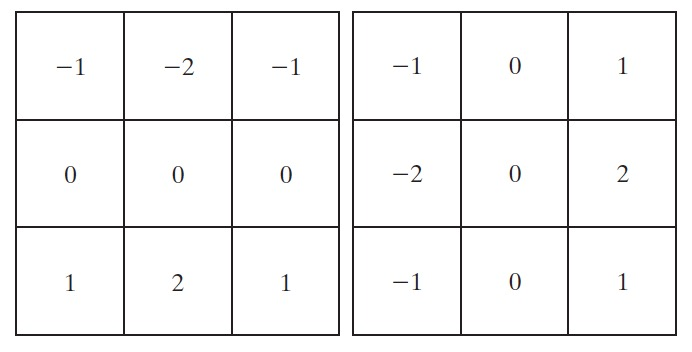Figure 36: Sobel Operator

$M(x,y) = \sqrt{\left((z_7 + 2 z_8 + z_9) - (z_1 + 2 z_2 + z_3) \right)^2 + \left((z_3 + 2 z_6 + z_9) - (z_1 + 2 z_4 + z_7) \right)^2}$

$M(x,y) = |(z_7 + 2 z_8 + z_9) - (z_1 + 2 z_2 + z_3)| + |(z_3 + 2 z_6 + z_9) - (z_1 + 2 z_4 + z_7)|$

## 3 形态学图像处理(Morphological Image Processing)

$$\mathbb{Z}^2$$ 中的集合可表示二值图像中的对象（集合的元素是图像中所有白色像素，或者所有黑色像素）。

### 3.1 数学形态学基本知识

#### 3.1.1 集合基本操作

(1) 子集。如果集合A中的每个元素又是另一个集合B中的一个元素，则称A为B的子集，表示为：
$A \subseteq B$
(2) 并集。如果某个集合C包含集合A和B中的所有元素，则称C为集合A和B的并集，表示为：
$C = A \cup B$
(3) 交集。如果某个集合D包含的元素同时属于集合A和B，则称D为集合A和B的交集，表示为：
$D = A \cap B$
(4) 补集。集合A的补集是不包含于集合A的元素所组成的集合，表示为：
$A^c = \{ w \mid w \notin A \}$
(5) 差集。集合A和B的差集是属于集合A，而不属于集合B的元素组成的集合，表示为：
$A-B = \{ w \mid w \in A, w \notin B \} = A \cap B^c$

#### 3.1.2 反射(Reflection)和平移(Translation)

$\hat{B} = \{ w \mid w = -b, b \in B \}$

$(B)_z = \{ c \mid c = b + z, b \in B \}$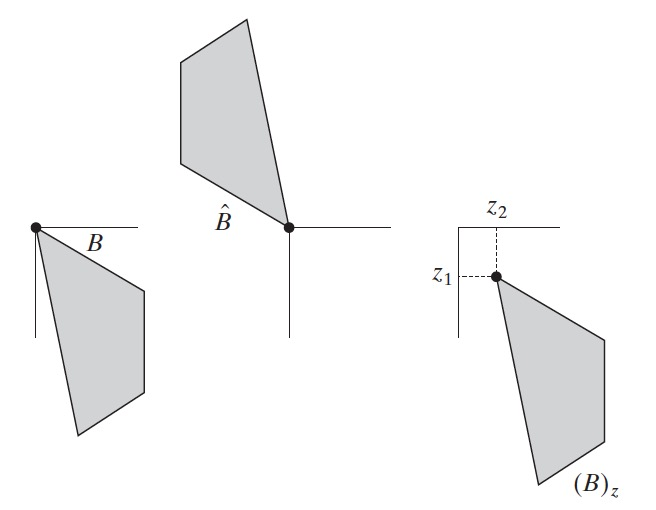Figure 37: 集合（图像）的反射和平移

#### 3.1.3 结构元(Structuring Element)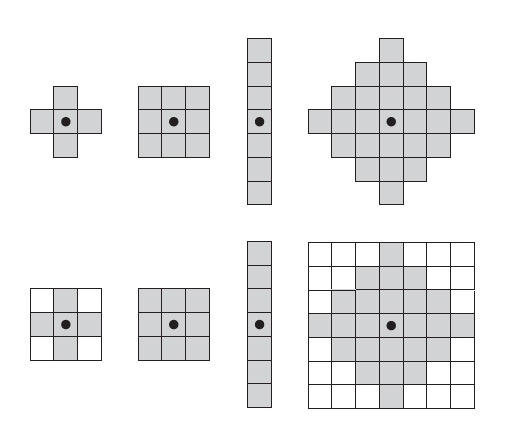Figure 38: 第一行为结构元例子，第二行是转换为矩形阵列的结构元。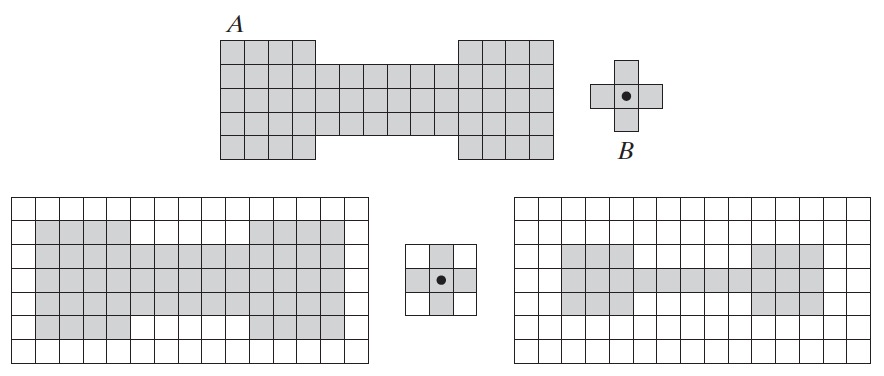Figure 39: 第一行分别是集合A和结构元B，第二行是：填充过的集合A，填充过的结构元B，由结构元B按约定规则处理A后形成的集合

### 3.2 腐蚀(Erosion)和膨胀(Dilation)

#### 3.2.1 腐蚀(Erosion)

A和B是 $$\mathbb{Z}^2$$ 中的集合，集合B对集合A的“腐蚀”定义为：
$A \ominus B=\{z \mid (B)_{z} \subseteq A\}$

$A \ominus B=\{z \mid (B)_z \cap A^c = \varnothing \}$

40 是用结构元B对图像A进行腐蚀的两个例子。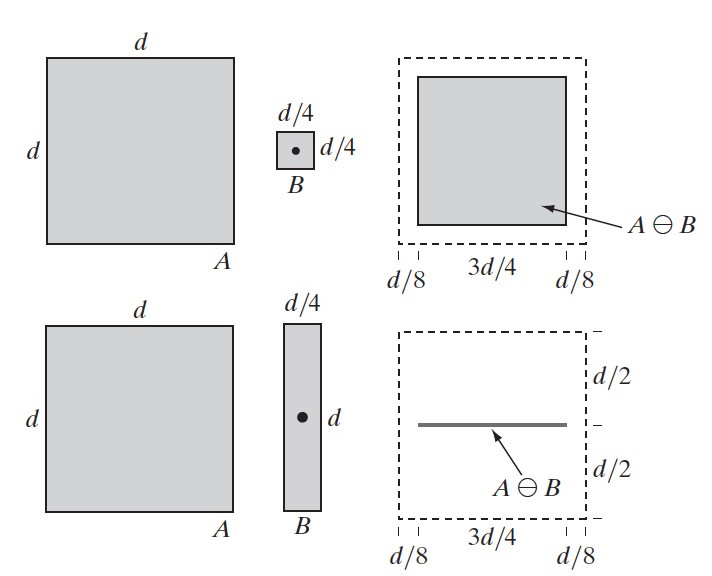Figure 40: 结构元B对图像A进行腐蚀的两个例子

41 是用形态相同但大小不同的结构元对同一图像进行腐蚀的例子。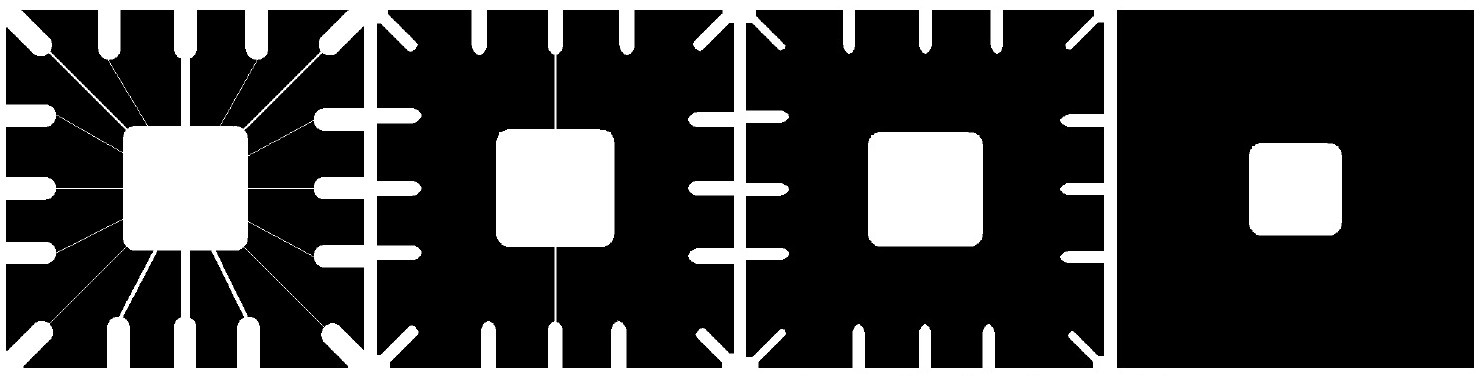Figure 41: 第一个是原图像(486x486)，后面三个图像分别用11x11，15x15，45x45的结构元（元素全为1）进行腐蚀处理后的结果

#### 3.2.2 膨胀(Dilation)

A和B是 $$\mathbb{Z}^2$$ 中的集合，集合B对集合A的“膨胀”定义为：
$A \oplus B=\{z \mid (\hat{B})_{z} \cap A \neq \varnothing \}$

42 是用结构元B（它是关于原点对称的，所以 $$\hat{B}=B$$ ）对图像A进行膨胀的两个例子。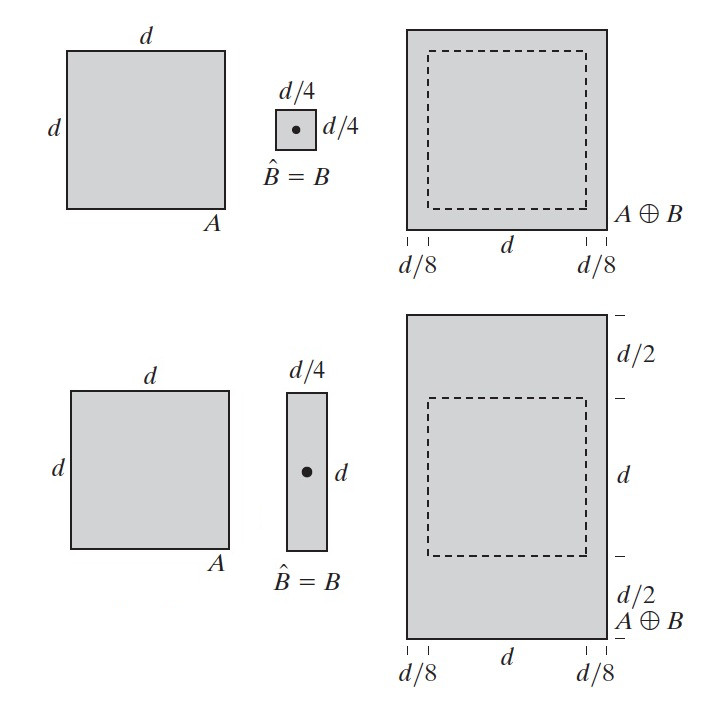Figure 42: 结构元B对图像A进行膨胀的两个例子

#### 3.2.3 腐蚀和膨胀的对偶性

\begin{aligned} (A \ominus B)^c = A^c \oplus \hat{B} \\ (A \oplus B)^c = A^c \ominus \hat{B} \end{aligned}

### 3.3 开操作(Opening)和闭操作(Closing)

#### 3.3.1 开操作（先腐蚀后膨胀）

$A\circ B=(A\ominus B) \oplus B$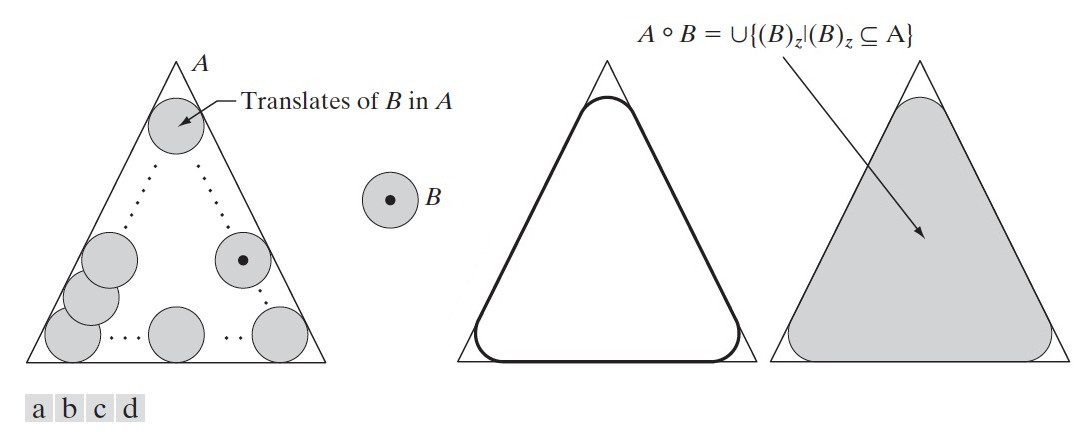Figure 43: (a)结构元B沿集合A的“内侧边界”滚动，(b)结构元，(c)粗线是开操作的外部边界，(d)阴影部分就是开操作 $$A\circ B$$ 的结果

$A\circ B= \bigcup \{ (B)_z \mid (B)_z \subseteq A \}$

#### 3.3.2 闭操作（先膨胀后腐蚀）

$A\bullet B=(A\oplus B) \ominus B$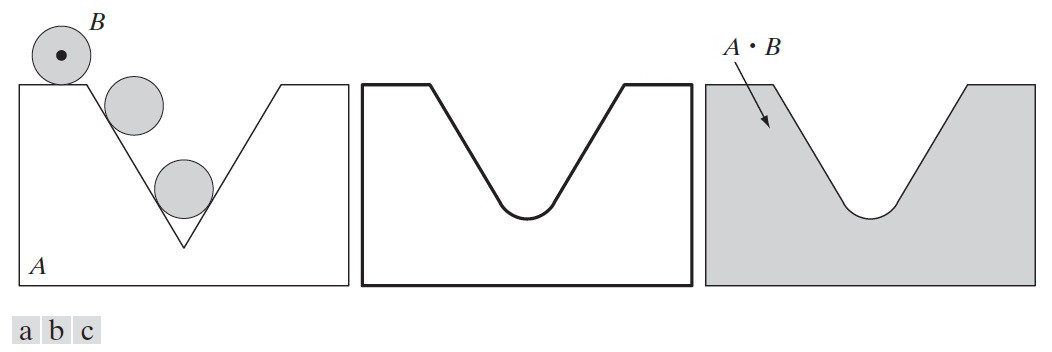Figure 44: (a) 结构元B沿集合A“外侧边界”滚动，(b)粗线是闭操作的外部边界，(d)阴影部分就是闭操作 $$A\bullet B$$ 的结果

#### 3.3.3 开操作和闭操作的对偶性

\begin{aligned} (A \circ B)^c = A^c \bullet \hat{B} \\ (A \bullet B)^c = A^c \circ \hat{B} \end{aligned}

#### 3.3.4 实例：腐蚀、膨胀、开操作、闭操作

45 是形态学基本操作（腐蚀、膨胀、开操作、闭操作）的实例。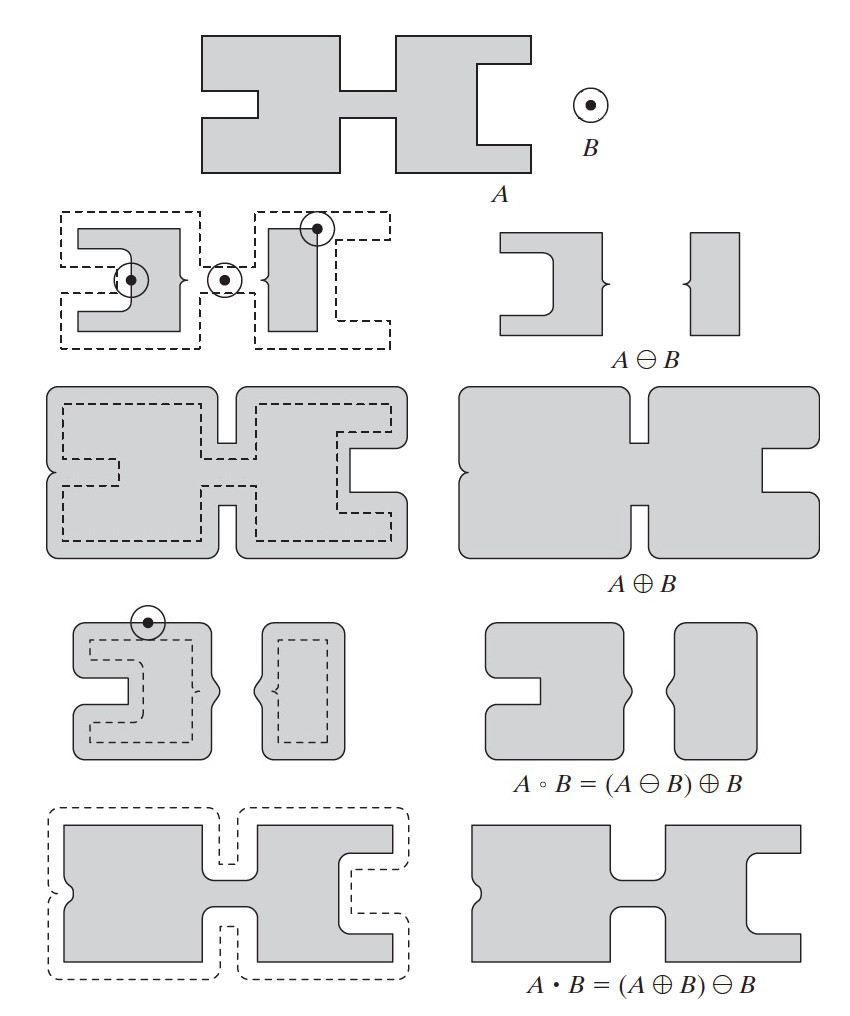Figure 45: 形态学基本操作（腐蚀、膨胀、开操作、闭操作）的实例

### 3.4 灰度级形态学

$\hat{b}(x,y) = b(-x, -y)$

#### 3.4.1 腐蚀和膨胀

$[f \ominus b] (x,y) = \min_{(s,t) \in b} \{ f(x+s, y+t) \}$

$[f \oplus b] (x,y) = \max_{(s,t) \in b} \{ f(x-s, y-t) \}$

#### 3.4.2 开操作和闭操作

$f \circ b = (f \ominus b) \oplus b$

$f \bullet B = (f \oplus b) \ominus b$

b对f的开操作的几何解释为： 在b的原点位置，从f的下表面垂直向上推动结构元b时，b的任何部分所达到的最高值。当b的原点访问f的每一个坐标 $$(x,y)$$ 所得到的所有值组成的集合就是b对f的开操作的结果。 闭操作的几何解释和开操作类似，只是“向上推”改为“向下推”，能达到的“最高值”改为“最低值”。

46 以一维的形式说明了灰度图像的开操作和闭操作的几何解释。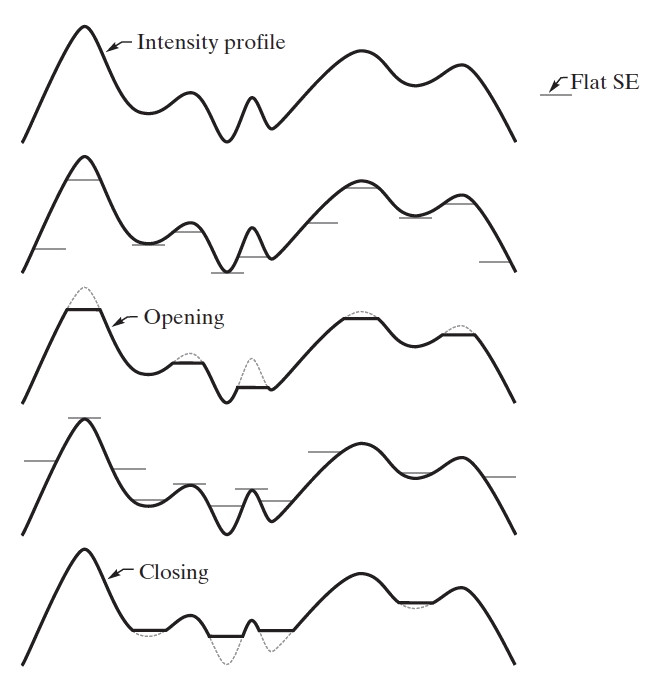Figure 46: 一维情形下的开操作和闭操作

##### 3.4.2.1 开操作和闭操作实例

47 是灰度图像开操作和闭操作实例。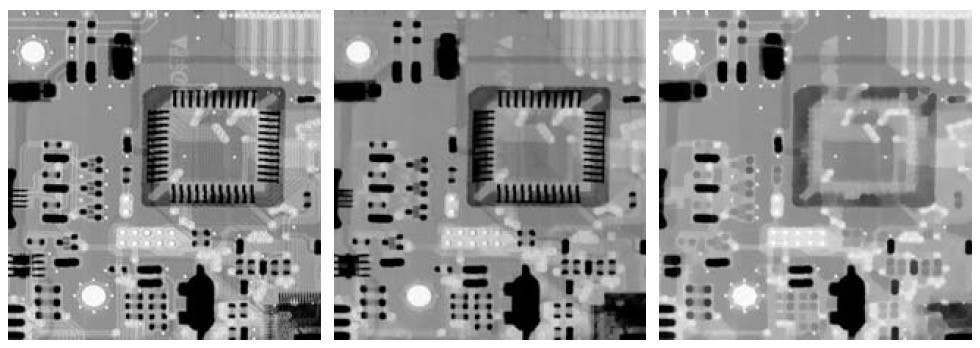Figure 47: 左为原图（448x425的灰度级图像），中图为使用半径为3像素的圆形结构元得到的开操作的结果（原图中较小的白点被去除了）；右图为使用半径为5像素的圆形结构元得到的闭操作的结果（原图中较小的黑点被去除了）

#### 3.4.3 一些基本的灰度级形态学算法

##### 3.4.3.1 形态学梯度(Morphological gradient)

$g = (f \oplus b) - (f \ominus b)$

48 是形态学梯度的一个实例。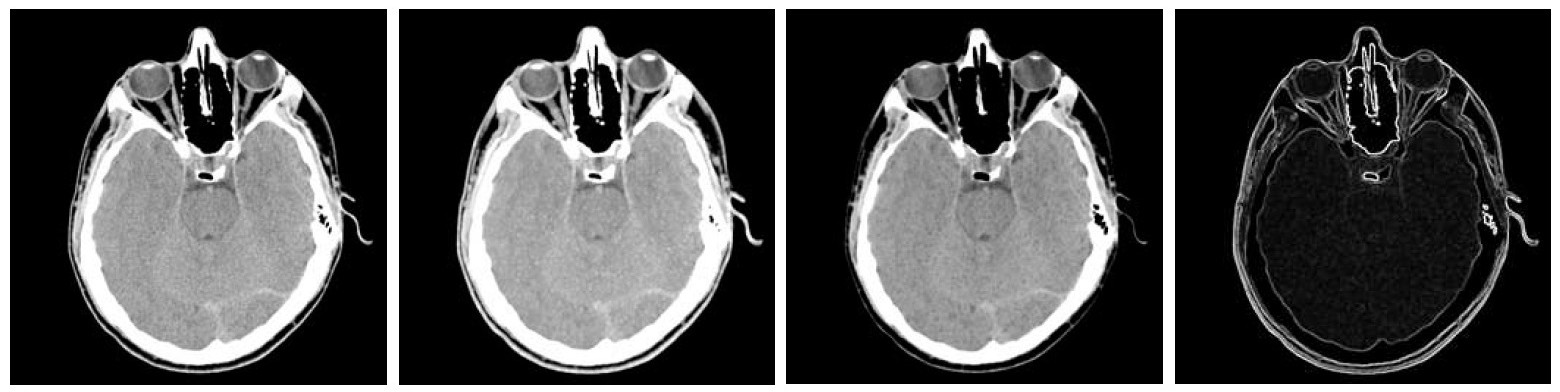Figure 48: 从左到右依次为原图（头部CT图像）、膨胀图、腐蚀图、形态学梯度图

##### 3.4.3.2 顶帽和底帽变换(Top-hat and bottom-hat transformation)

$T_{hat}(f) = f - (f \circ b)$

$B_{hat}(f) = (f \bullet b) - f$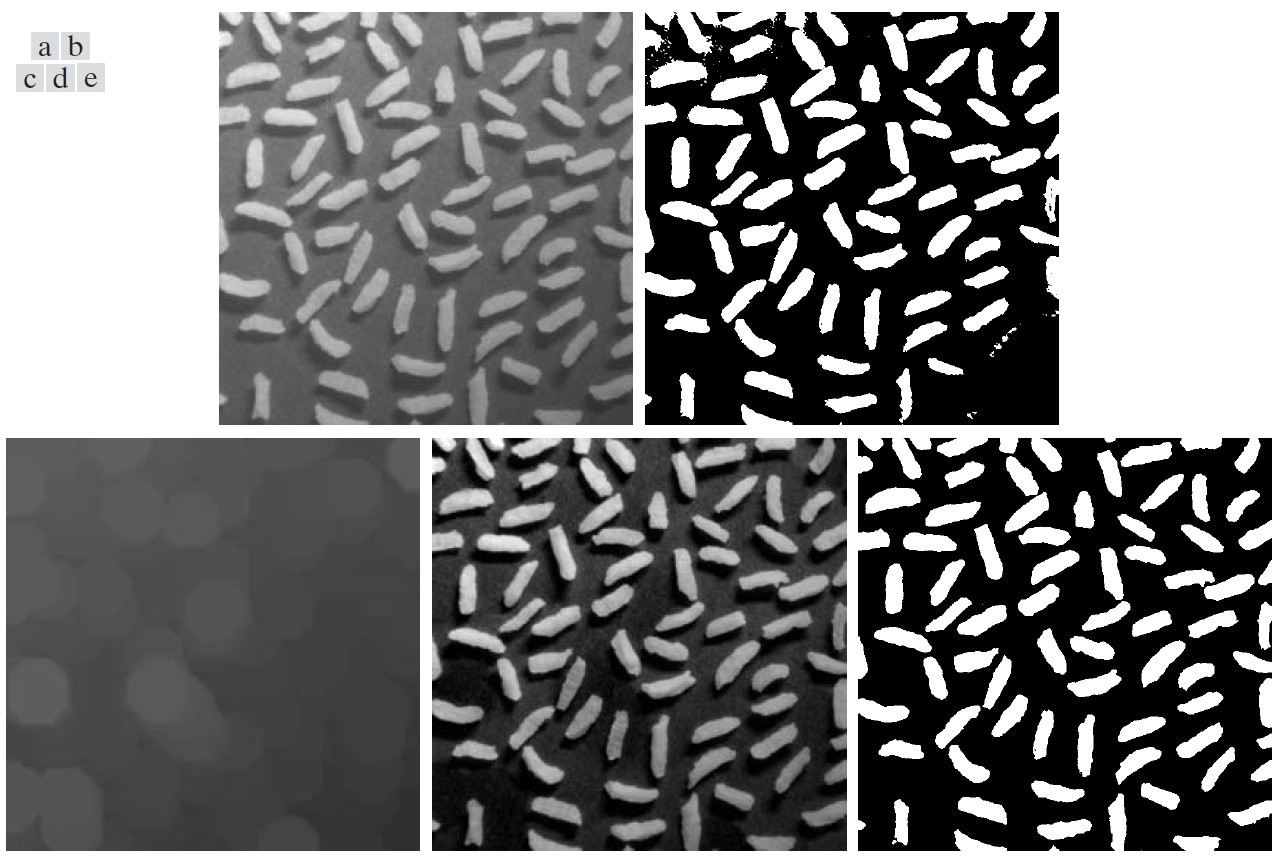Figure 49: (a)大小为600x600的原图（对比度低），(b)直接进行阈值处理（右下角出现了错误），(c)进行开操作的图像（结构元为半径为50的圆，结果只剩下了背景），(d)顶帽变换后的图像（对比度高），(e)对(d)图进行阈值处理（不再有错误）

## 4 图像分割(Image Segmentation)

### 4.1 孤立点、线、边缘的检测

#### 4.1.1 孤立点的检测（拉普拉斯算子）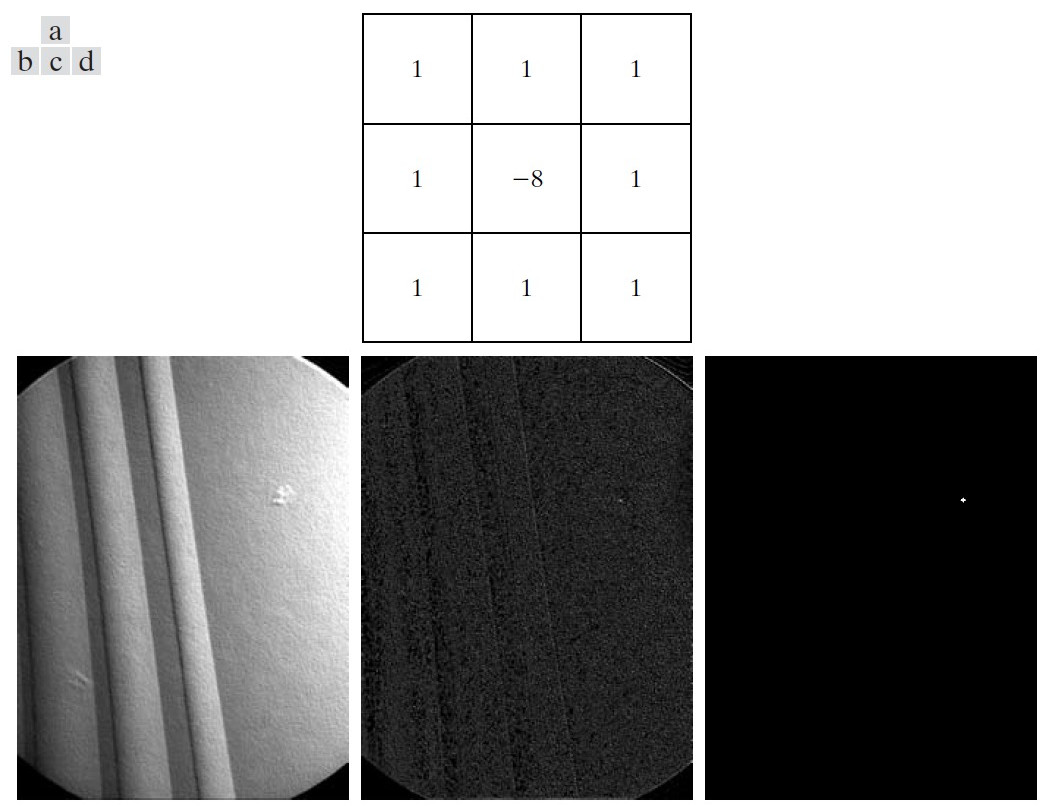Figure 50: (a)点检测（拉普拉斯）模板，(b)原图像（带有一个通孔的涡轮叶片X射线图），(c)模板和图像卷积的结果，(d)对(c)图阈值处理后的二值图像（找到了一个点）

#### 4.1.2 线的检测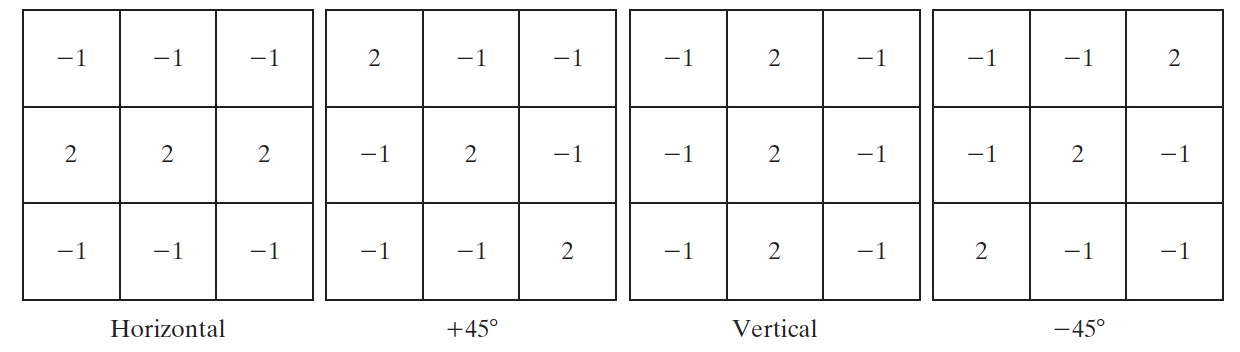Figure 51: 线检测模板。角度是相对于图 1 中的坐标轴系统

#### 4.1.3 边缘检测原理：一阶导数和二阶导数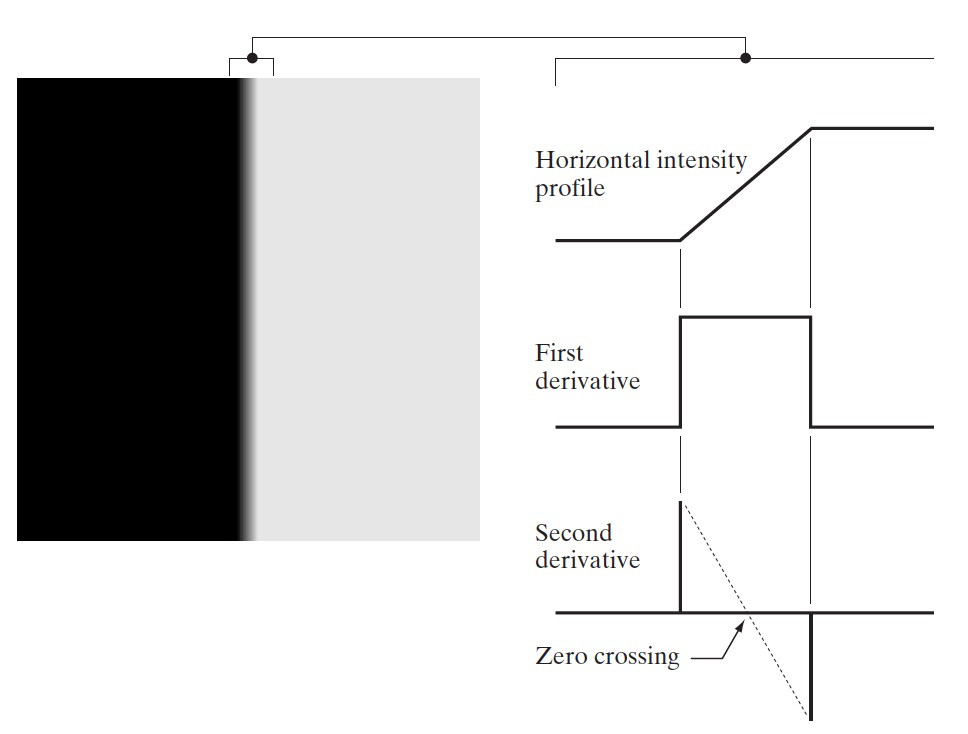Figure 52: 左图被界线明显地分为两个区域，右图是界线的剖面图及其一阶导数和二阶导数

#### 4.1.4 基本的边缘检测：梯度算子(Prewitt, Sobel, Kirsch Compass, Robinson Compass)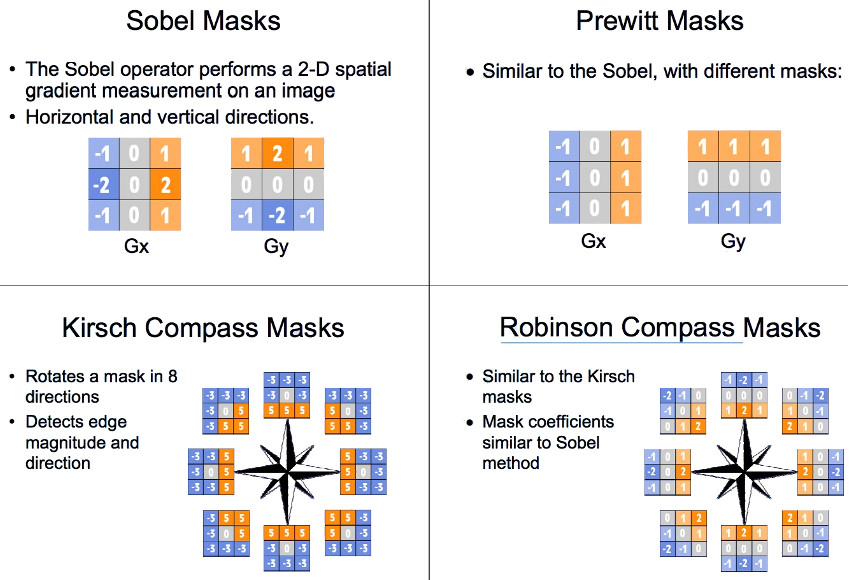Figure 53: 常见的边缘检测梯度算子（摘自：http://www.cs.tau.ac.il/~turkel/notes/sem.pdf

#### 4.1.5 先进的边缘检测：Marr-Hildreth edge detector (LoG, DoG)

2.5.2 中介绍过，使用二阶微分可以提取出图像中灰度变化较快的部分。但是，直接对图像使用拉普拉斯算子 $$\nabla^2 f = \frac{\partial^2 f}{\partial x^2} + \frac{\partial^2 f}{\partial y^2}$$ ，得到的结果中图像的噪声很可能被放大。

Marr-Hildreth边缘检测算法 总结如下：

$g(x,y) = \nabla^2 [G(x,y) \star f(x,y)]$

##### 4.1.5.1 Laplacian of Gaussian(LoG, Mexican hat operator)

$g(x,y) = [ \nabla^2 G(x,y) ] \star f(x,y)$

$G(x,y) = e^{- \frac{x^2 + y^2}{2 \sigma^2}}$

$\frac{\partial}{\partial x} G(x,y) = \frac{\partial}{\partial x} e^{- (x^2 + y^2)/2 \sigma^2} = - \frac{x}{\sigma^2} e^{- (x^2 + y^2)/2 \sigma^2}$

$\frac{\partial^2}{\partial x^2} G(x,y) = \frac{x^2}{\sigma^4} e^{- (x^2 + y^2)/2 \sigma^2} - \frac{1}{\sigma^2} e^{- (x^2 + y^2)/2 \sigma^2} = \frac{x^2 - \sigma^2}{\sigma^4} e^{- (x^2 + y^2)/2 \sigma^2}$

\begin{aligned} \nabla^2 G(x,y) & = \frac{\partial^2}{\partial x^2} G(x,y) + \frac{\partial^2}{\partial y^2} G(x,y) \\ & = \frac{x^2 - \sigma^2}{\sigma^4} e^{- (x^2 + y^2)/2 \sigma^2} + \frac{y^2 - \sigma^2}{\sigma^4} e^{- (x^2 + y^2)/2 \sigma^2} \\ & = \frac{x^2 + y^2 - 2 \sigma^2}{\sigma^4} e^{- (x^2 + y^2)/2 \sigma^2} \\ \end{aligned}

$\text{LoG}(x,y) \triangleq \nabla^2 G(x,y) =\frac{x^2+y^2-2\sigma^2}{\sigma^4} e^{-\frac{(x^2+y^2)}{2\sigma^2}}$

LoG的负函数的三维图如图 54 (a)所示，其形状像个帽子，有时 LoG函数也被称为墨西哥草帽算子(Mexican hat operator)。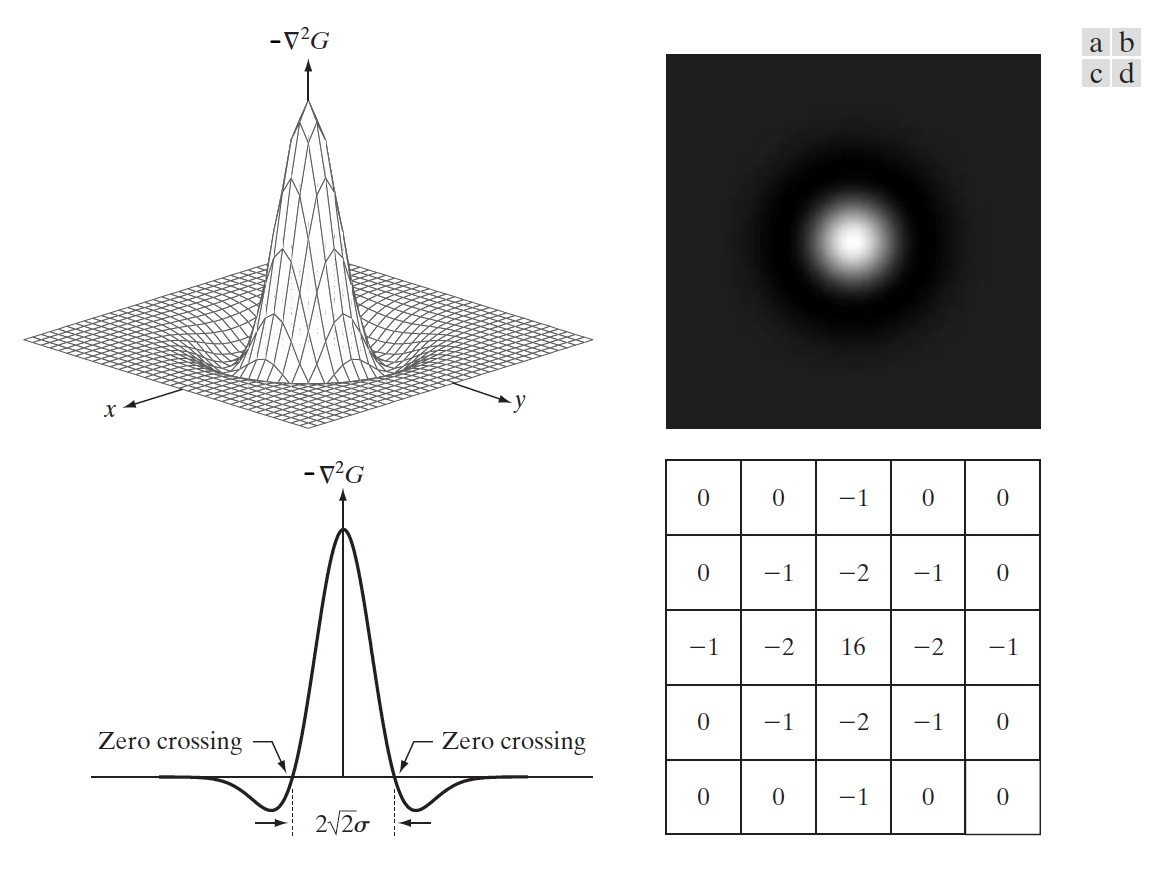Figure 54: (a)负LoG的三维图，(b)以图像形式显示负LoG，(c)图(a)的横截面（注意零交叉点），(d)负LoG的一个 $$5\times5$$ 离散近似模板

##### 4.1.5.2 Difference of Gaussian(DoG)

$\text{LoG}(x,y) \approx \text{DoG}(x,y) = \frac{1}{2 \pi \sigma_1^2} e^{- \frac{(x^2 + y^2)}{2 \sigma_1^2}} - \frac{1}{2 \pi \sigma_2^2} e^{- \frac{(x^2 + y^2)}{2 \sigma_2^2}}$

$\sigma^2 = \frac{\sigma_1^2 \sigma_2^2}{\sigma_1^2 - \sigma_2^2} \ln \left[ \frac{\sigma_1^2}{\sigma_2^2} \right]$

#### 4.1.6 更先进的边缘检测：Canny edge detector

Canny edge detector是目前讨论过的边缘检测算法中最优秀的。

Canny算法分为如下几个步骤：
(1) 用高斯滤波器平滑输入图像；
(2) 计算梯度幅值图像和梯度角度图像；
(3) 对梯度幅值图像应用“非最大抑制(Non-maximum suppression)”算法；
(4) 用“双阈值处理(Double threshold)”和来检测边缘；
(5) 用特征综合方法收集来自多尺度的最终的边缘信息（不过，通常的算法实现都省略了这一步）。

“双阈值处理”的目的是减少伪边缘点，其具体过程为：设置两个阈值，上限和下限。如果一个像素的梯度大于上限阈值，则认为是边缘像素；如果低于下限阈值，则不认为是边缘像素，被抛弃；如果介于二者之间，只有当其“与高于上限阈值的像素连接”时才被接受。

55 是用不同算法对同一图像进行边缘检测的例子。从这个例子可知Canny算法的效果最好。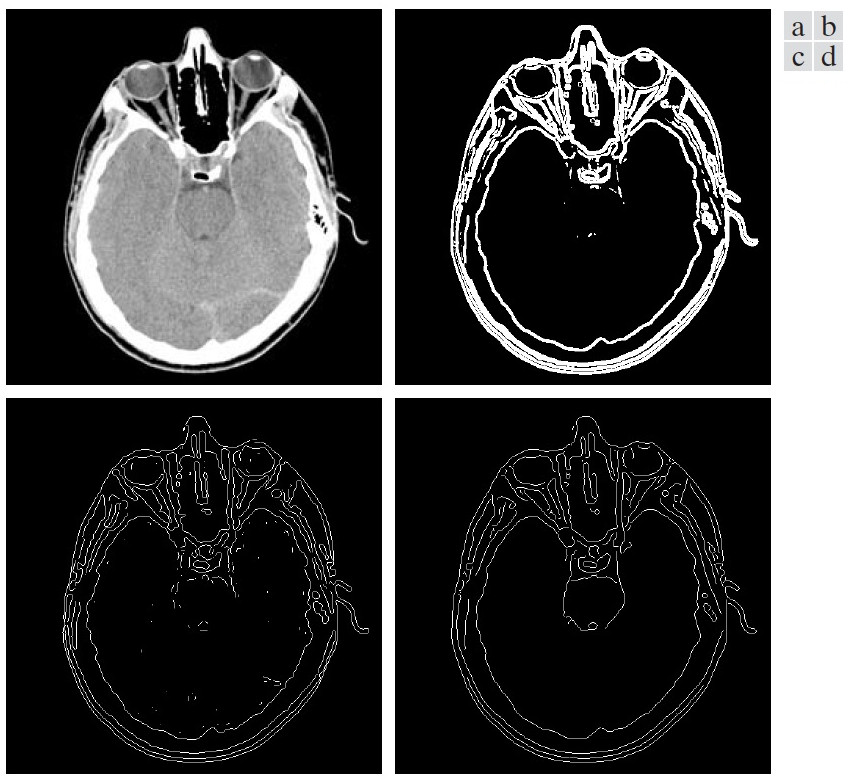Figure 55: (a)原始的头部CT图像，(b)阈值处理后的梯度图像，(c)用Marr-Hildreth算法得到的图像，(d)用Canny算法得到的图像

##### 4.1.6.1 非最大抑制(Non-maximum suppression)

$M(x,y) = \sqrt{(\frac{\partial f_s}{\partial x})^2 + (\frac{\partial f_s}{\partial y})^2}$

$\alpha(x,y) = \arctan \left[ \frac{\frac{\partial f_s}{\partial y}}{\frac{\partial f_s}{\partial x}} \right]$

(1) 把“和区域中心点的梯度角度 $$\alpha(x,y)$$ 最接近的方向”记为 $$d_k$$ （前面介绍的四个方向之一）；
(2) 在 $$M(x,y)$$ 图像中，如果区域中心点的值大于其沿着 $$d_k$$ 方向的两个相邻点，则保留下来（即 $$g_N(x,y) = M(x,y)$$ ），否则就抑制它（即 $$g_N(x,y) = 0$$ ）。这就是“非最大抑制”算法名字的由来。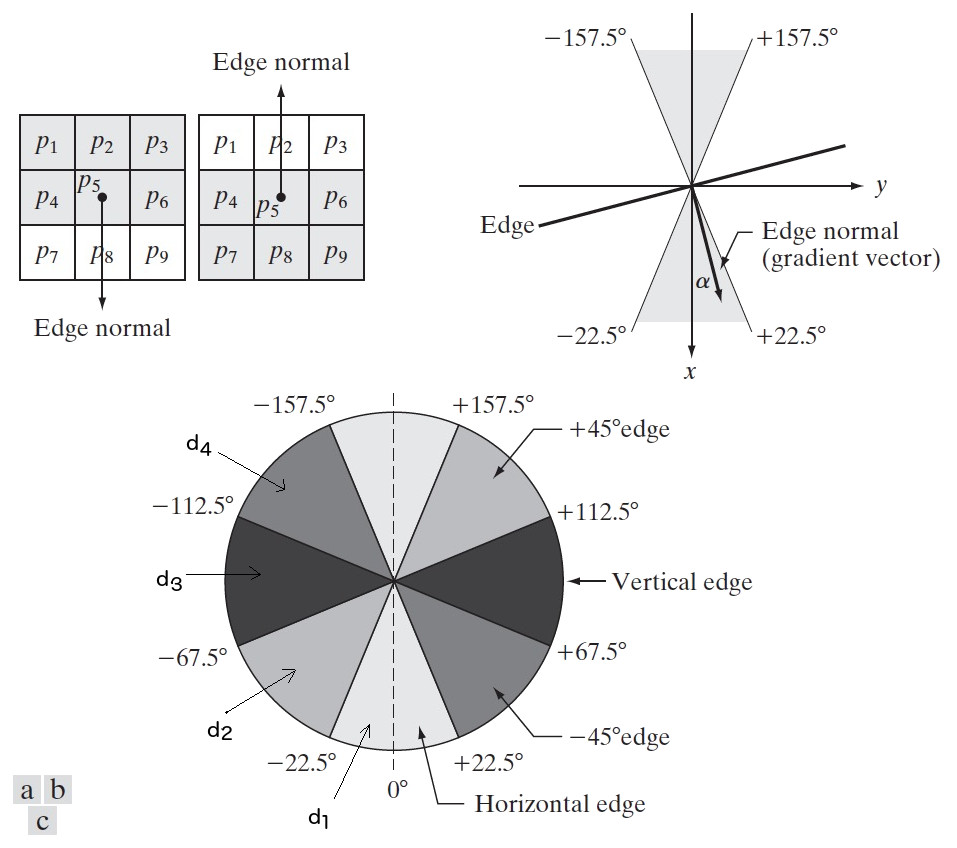Figure 56: 非最大抑制（normal是“法线”的意思）

#### 4.1.7 用霍夫变换(Hough Transform)检测形状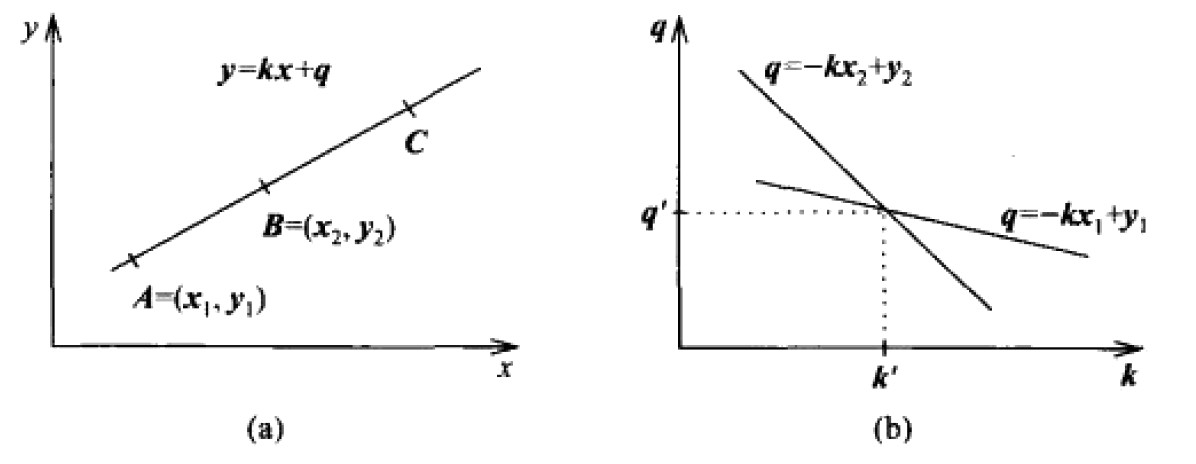Figure 57: Hough变换原理。(a)原图像空间，(b)参数空间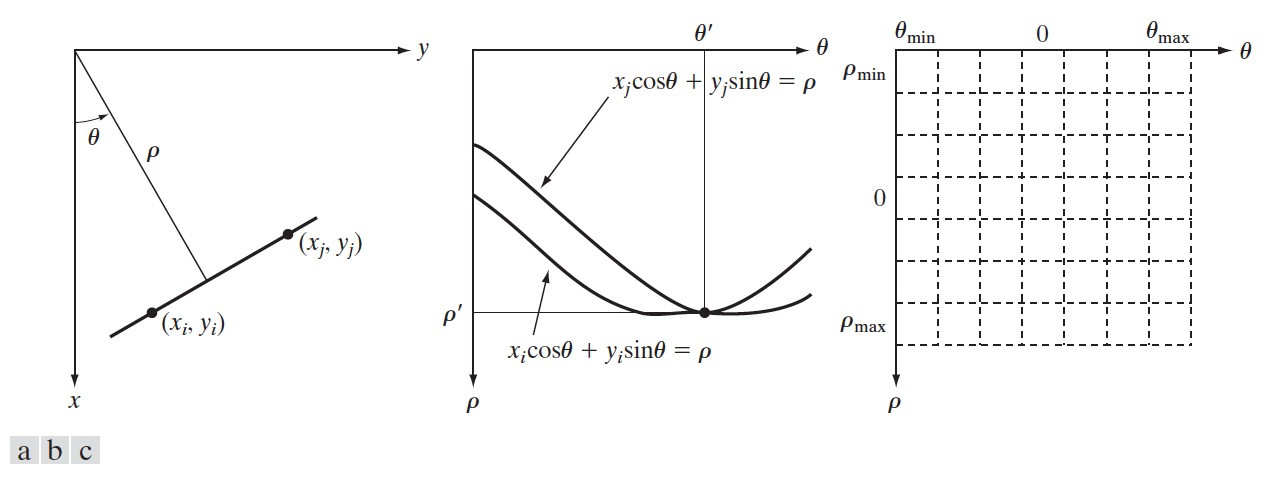Figure 58: (a)原图像空间，(b)参数空间 $$\rho, \theta$$ ，(c)把 $$\rho, \theta$$ 空间划分为累加单元（某个累加单元的点非常多时就说明找到了原图像空间的直线）

##### 4.1.7.1 霍夫变换对噪声不敏感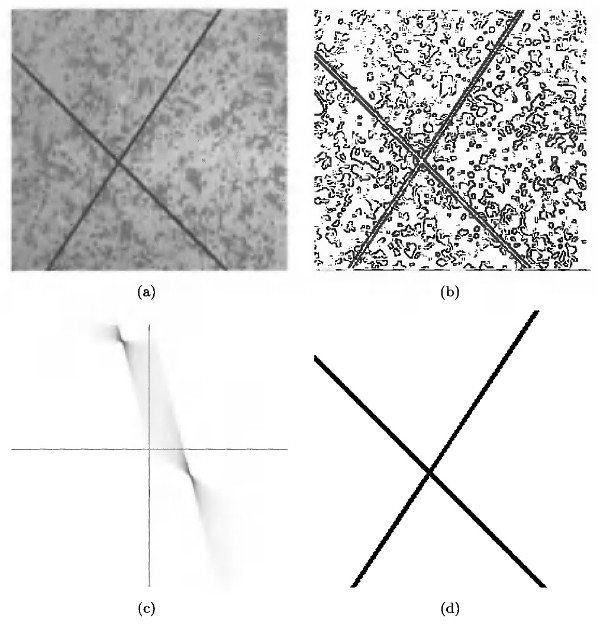Figure 59: (a)原始图像，(b)边缘图像，(c)Hough变换的参数空间，(d)Hough变换检测到的直线

### 4.2 阈值处理(Thresholding)

#### 4.2.1 阈值处理基础知识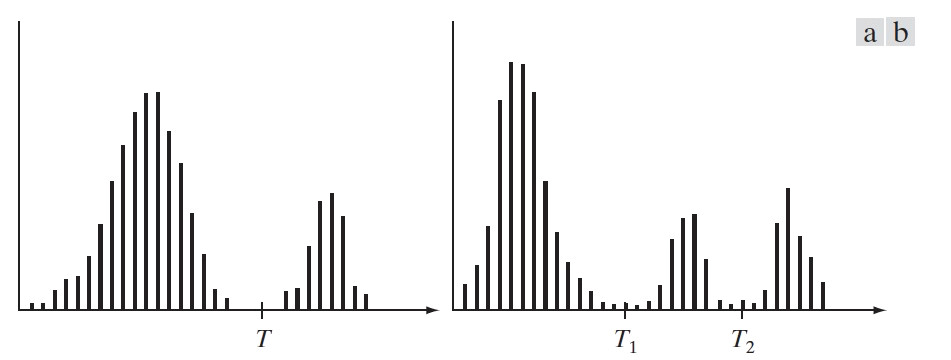Figure 60: (a)可被单阈值分隔的灰度直方图，(b)可被双阈值分隔的灰度直方图

$g(x,y) = \begin{cases} 1, & f(x,y) > T \\ 0, & f(x,y) \le T \end{cases}$

##### 4.2.1.1 噪声对阈值处理的影响

61 显示了噪声是如何影响一幅图像的直方图的。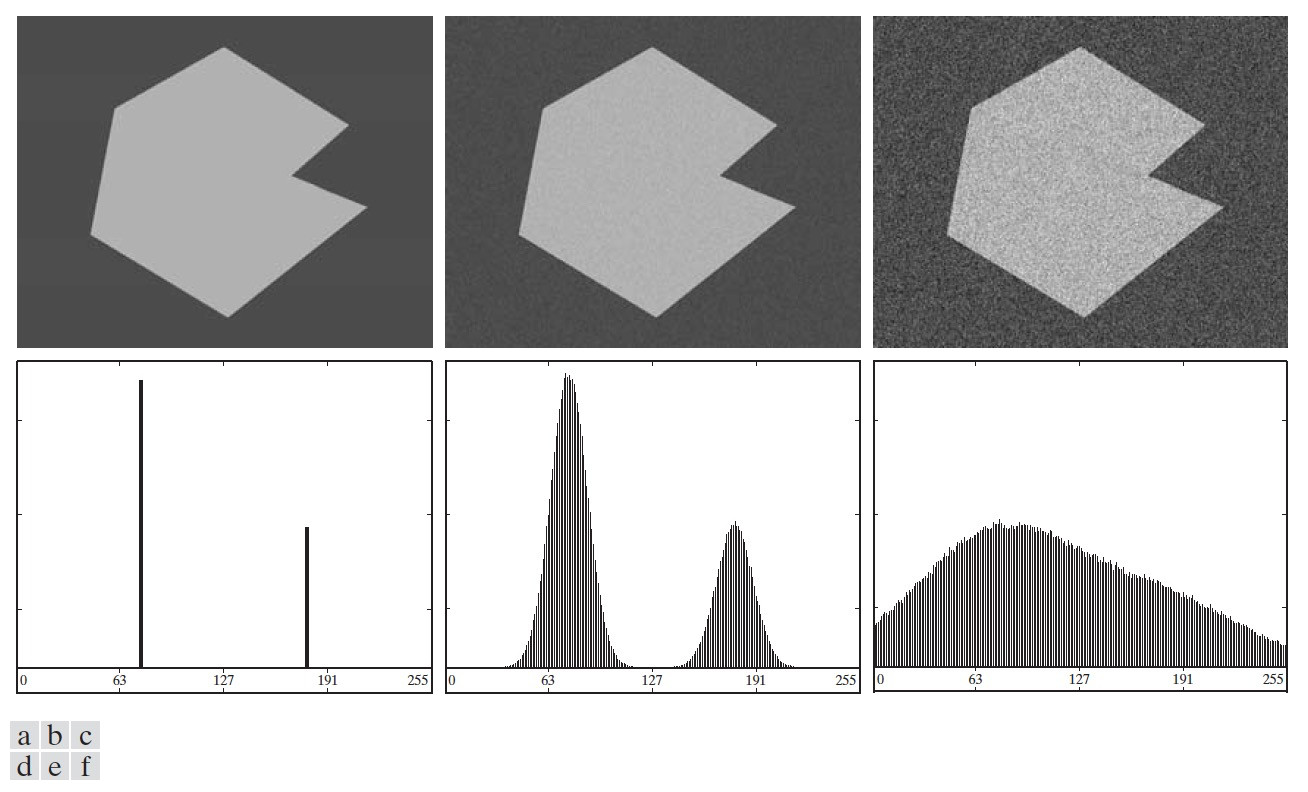Figure 61: (a)无噪声图像，(b)带有均值为0，标准差为10个灰度级的加性高斯噪声的图像，(c)带有均值为0，标准差为50个灰度级的加性高斯噪声的图像，(d)~(f)相应的直方图

61 (a)和(b)可以用阈值分割其背景和物体，但图 61 (c)中的噪声污染过于严重，无法直接使用阈值处理来分割图像的背景和物体了。这时，我们可以尝试 4.2.44.2.5 中介绍的方法来分割其背景和物体。

##### 4.2.1.2 光照和反射对阈值处理的影响

62 (c)显示了非均匀光照对一幅图像的直方图的影响，我们无法直接使用阈值处理来分割它的背景和物体。如果光照是均匀的，但图像的反射不均匀也会得到类似的结果。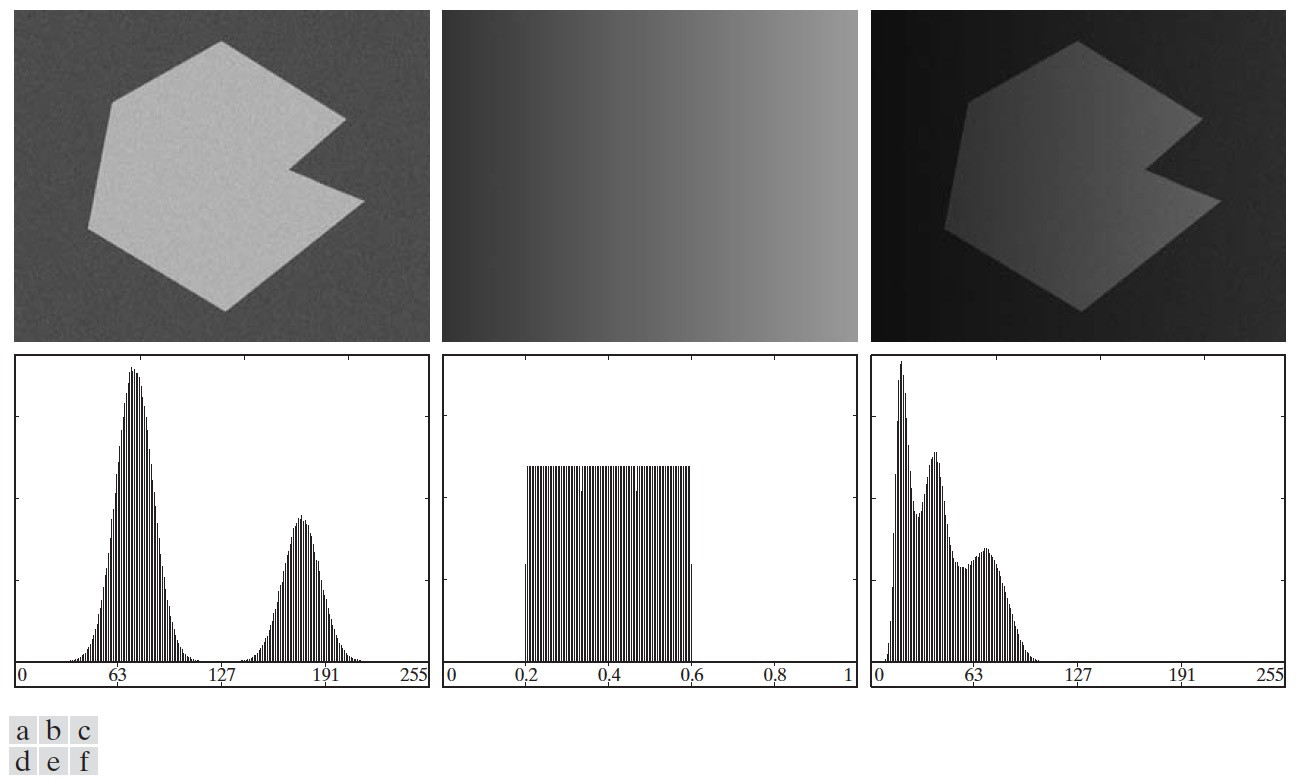Figure 62: (a)带有噪声的图像，(b)一个灰度斜坡图像，(c)图(a)和图(c)相乘的图像（可模拟非均匀光照图像），(d)~(f)相应的直方图

(1) 直接校正这种阴影模式。例如，非均匀光照可以用相反的模式与图像相乘来校正；
(2) 可尝试使用 3.4.3.2 中介绍的顶帽变换来校正全局阴影模式；
(3) 可尝试使用 4.2.6 中介绍的可变阈值处理。

#### 4.2.2 基本全局阈值处理

(1) 为全局阈值 $$T$$ 选择一个初始估计值；
(2) 用阈值 $$T$$ 分割该图像。这将产生两组像素： $$G_1$$ 由灰度值大于 $$T$$ 的所有像素组成， $$G_2$$ 由灰度值小于等于 $$T$$ 的所有像素组成。
(3) 对 $$G_1$$ 和 $$G_2$$ 的像素分别计算平均灰度值，记为 $$m_1$$ 和 $$m_2$$ 。
(4) 计算一个新的阈值：
$T = \frac{1}{2} (m_1 + m_2)$
(5) 重复步骤2到步骤4，直到连续迭代中的 $$T$$ 值间的差小于一个预定义的参数 $$\Delta T$$ 为止。

63 是全局阈值处理的一个例子。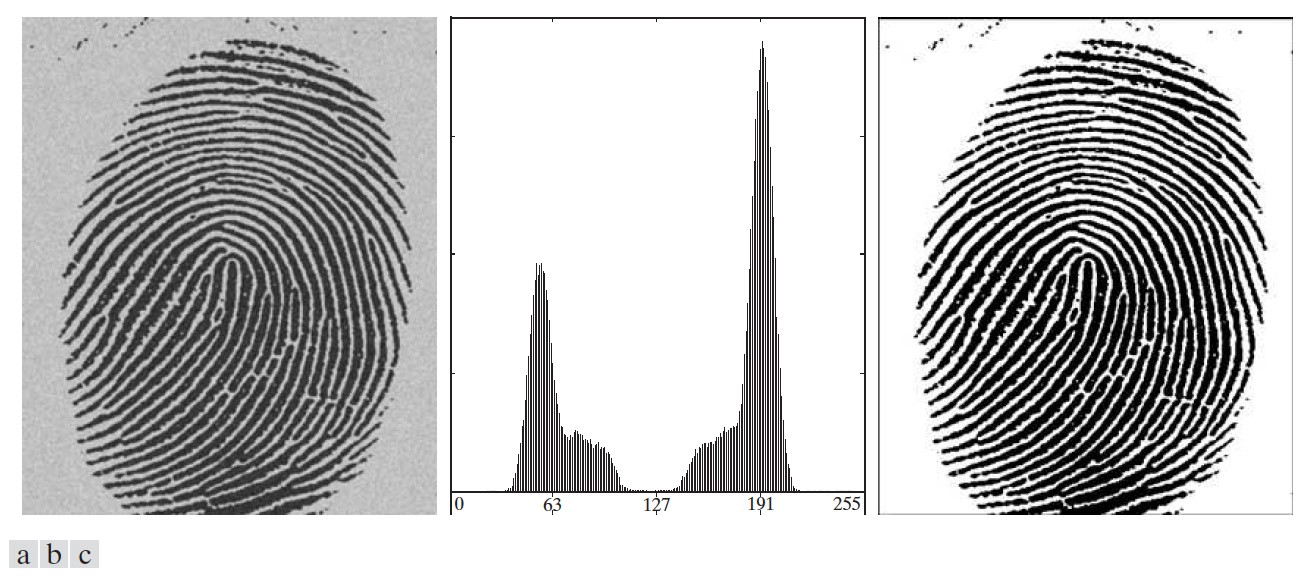Figure 63: (a)带噪声的指纹，(b)图(a)的直方图，(c)使用全局阈值处理的结果（迭代后得到的阈值 $$T$$ 为125.4）

#### 4.2.3 用Otsu方法（类间最大方差法）进行全局阈值处理

Otsu方法的基本思想为：按图像的灰度特性，将图像分成背景和物体二个部分时，背景和目标之间的方差越大，说明图像的这二个部分的差别会越大，分类效果越好。 但怎么描述背景和目标之间的方差（称为类间方差）呢？请看下文说明。

$P_1(k) = \sum_{i=0}^{k} p_i$

$P_2(k) = 1 - P_1(k)$

$\sigma_G^2 = \sum_{i=0}^{L-1} (i - m_G)^2 p_i$

${\color{red}{\sigma_B^2 = P_1 (m_1 - m_G)^2 + P_2 (m_2 - m_G)^2}}$

$\sigma_B^2 = P_1 P_2 (m_1 - m_2)^2$

$$C_1$$ 和 $$C_2$$ 类是以阈值 $$k$$ 为分类依据的， $$k$$ 取不同的值时，可得到不同的类间方差 $$\sigma_B^2(k)$$ ， Otsu方法的核心 就是求当 $$\sigma_B^2(k)$$ 取得最大值时，对应的阈值 $$k^{*}$$ 作为最终的阈值。用公式表达就是：
$\sigma_B^2(k^*) = \max_{0 \le k \le L-1} \sigma_B^2(k)$

##### 4.2.3.1 可分性度量

$\eta(k) = \frac{\sigma_B^2(k)}{\sigma_G^2}$

$$0 \le \eta(k) \le 1$$ ，当 $$\eta(k)$$ 越接近于1时，说明阈值 $$k$$ 的可分性越好。

##### 4.2.3.2 Otsu方法实例

64 是用基本全局阈值处理（参见节 4.2.2 ）和用Otsu方法进行全局阈值处理的实例，显然Otsu方法得到的结果更好。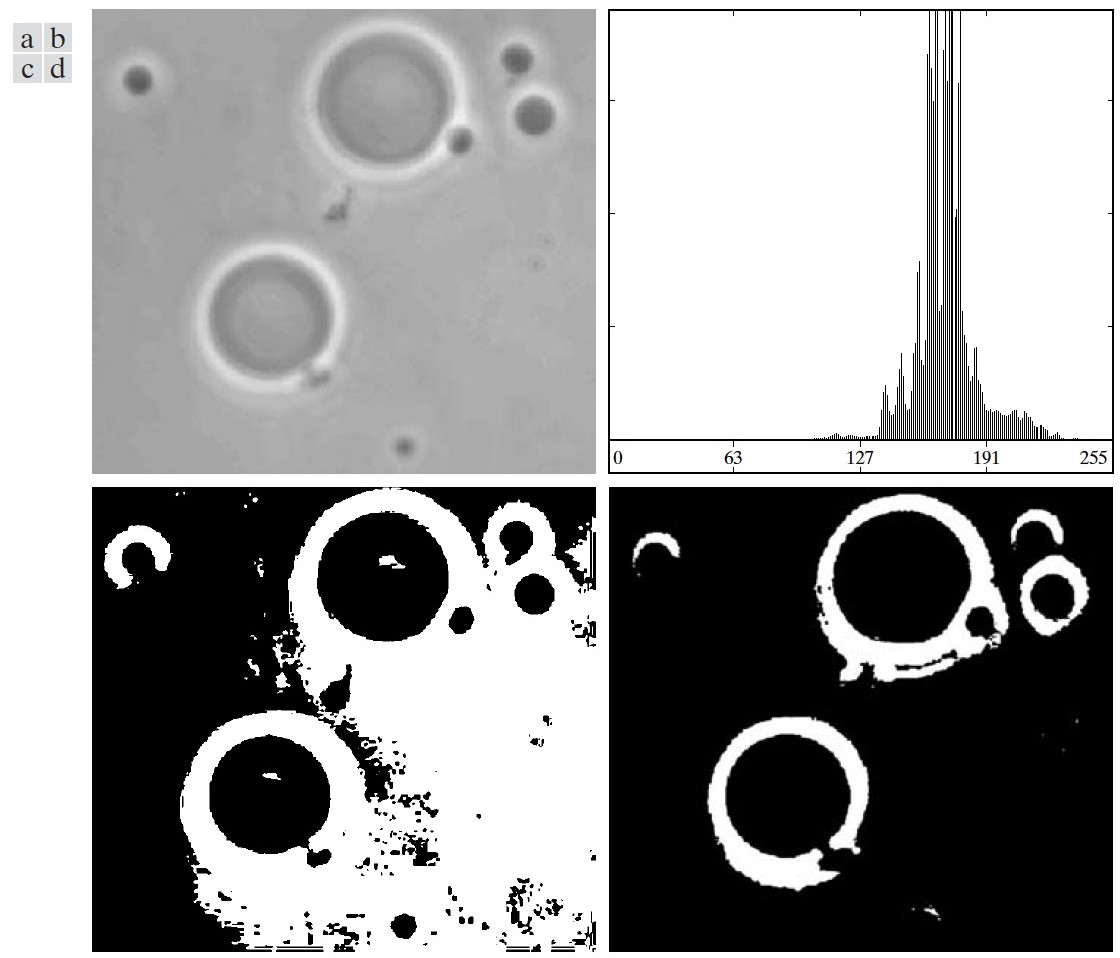Figure 64: (a)原图像，(b)原图像直方图，(c)基本全局阈值处理（得到的阈值为169），(d)Otsu方法（得到的阈值为181，对应的可分性度量 $$\eta=0.467$$ ）

#### 4.2.4 利用图像平滑改善全局阈值处理

65 (a)是一个被噪声污染得比较严重的图像，其直方图中已经找不到不同的波峰，用基本全局阈值得或Otsu方法都无法成功分割背景和物体。这时，可以尝试在全局阈值处理前，先对原图像进行平滑处理。如图 65 (c)是对(a)图使用 $$5\times5$$ 均值模板进行平滑处理后的结果，这时直方图已经出现了不同的波峰，可以使用全局阈值处理来分割背景和物体了。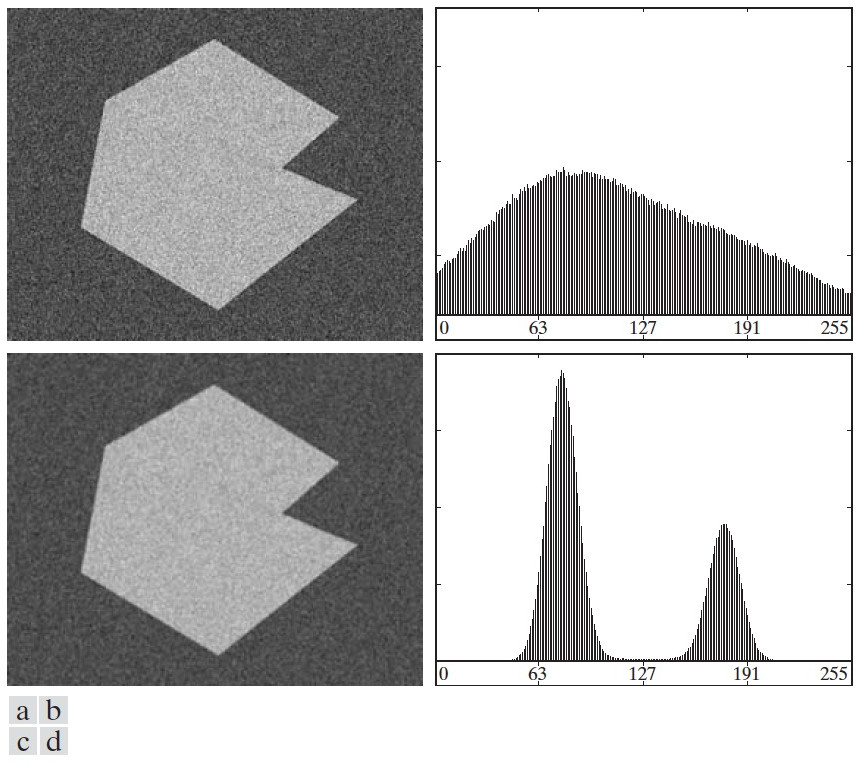Figure 65: (a)噪声图像，(b)图(a)直方图，(c)对图(a)使用 $$5\times5$$ 均值模板进行平滑处理后的结果，(d)图(c)的直方图

#### 4.2.5 利用边缘改善全局阈值处理

4.2.4 中介绍的利用平滑方法并不能改善所有的全局阈值处理，如图 66 所示，背景中的物体太小，经过平滑处理后，没有产生新的波峰，还是无法使用全局阈值处理。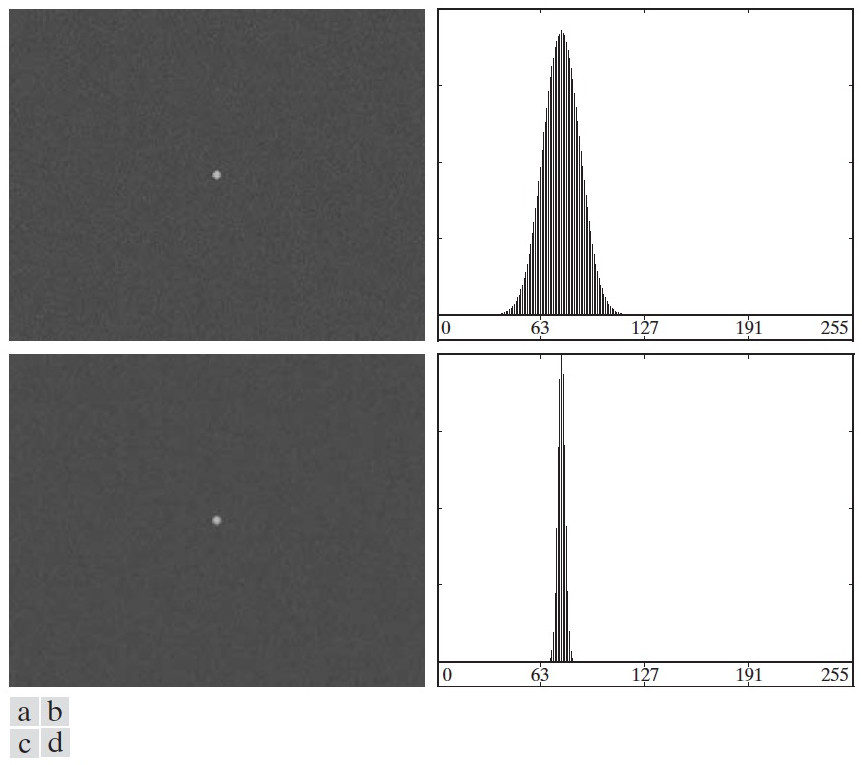Figure 66: (a)原始图像，(b)图(a)直方图，(c)对图(a)使用 $$5\times5$$ 均值模板进行平滑处理后的结果，(d)图(c)的直方图（还是没有产生新的波峰）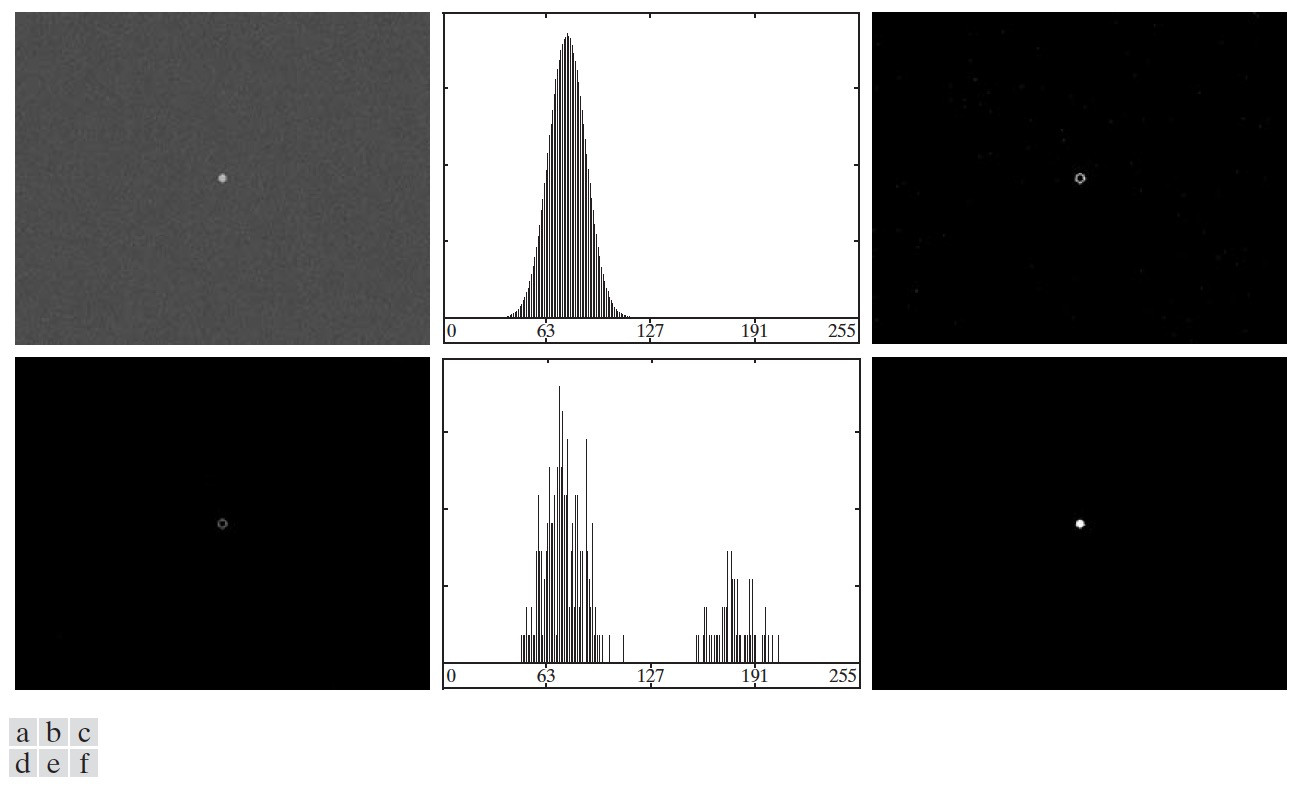Figure 67: (a)原始图像，(b)图(a)直方图，(c)梯度图像（阈值处理为二值图像），(d)图(a)和图(c)的对应像素相乘（非零像素构成的子图是原始图像中位于或靠近的像素点），(e)图(d)中非零像素的直方图，(f)用Otsu方法处理直方图(e)，得到阈值（为134）后，用该阈值处理原始图像的结果

#### 4.2.6 可变阈值处理（局部阈值处理/区域阈值处理/动态阈值处理/自适应阈值处理）

##### 4.2.6.1 图像分块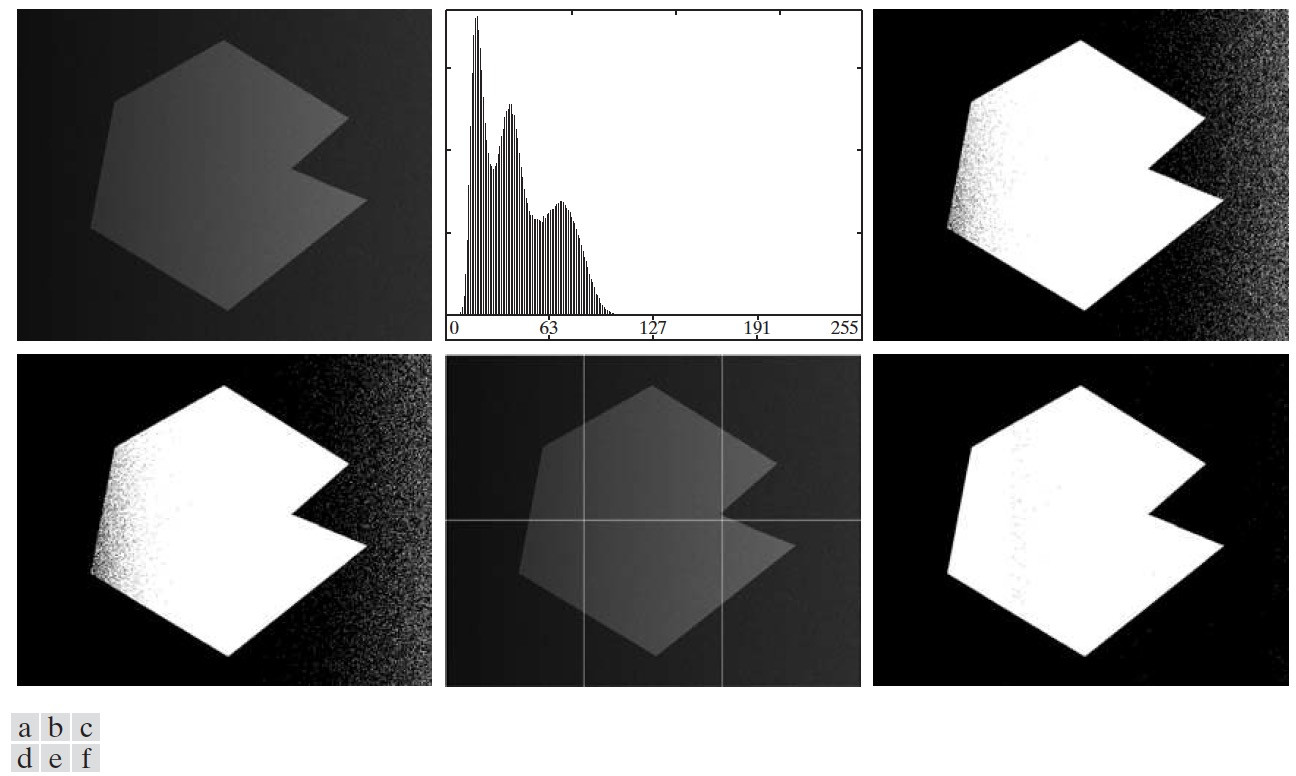Figure 68: (a)带噪声阴影的图像，(b)图(a)的直方图，(c)用基本全局阈值处理的结果，(d)用Otsu方法得到的结果，(e)把图(a)分为6个子图像，(f)对图(e)每一幅子图像应用Otsu方法的结果

68 (e)的6个子图像的直方图如图 69 所示，显然对每个图像直接用阈值处理即可分割背景和物体了。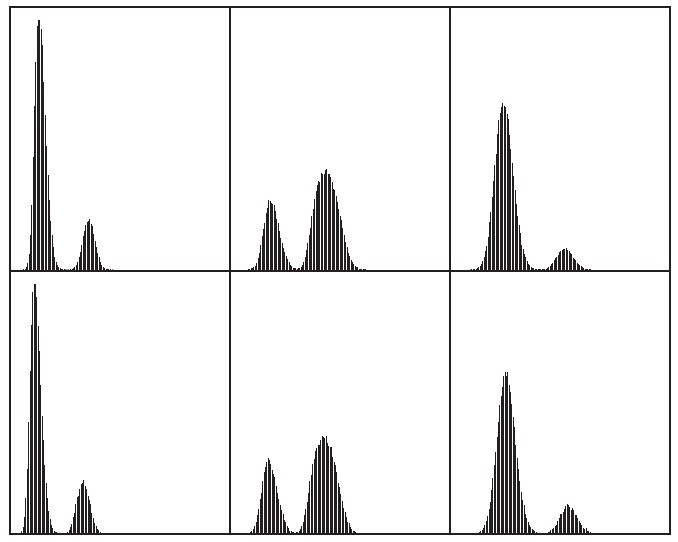Figure 69: 68 (e)的6个子图像的直方图

##### 4.2.6.2 基于局部图像特性的可变阈值处理

$T_{xy} = a \sigma_{xy} + b m_{xy}$

$g(x,y) = \begin{cases} 1, & f(x,y) > T_{xy} \\ 0, & f(x,y) \le T_{xy} \end{cases}$

##### 4.2.6.3 使用移动平均(Using moving average)

“移动平均(Moving average)”采用下面方式计算 $$m_{xy}$$ ：把图像以Z字形展开为一维序列，表示为 $$z_1, z_2, \cdots$$ ，图 70 是移动平均的原理。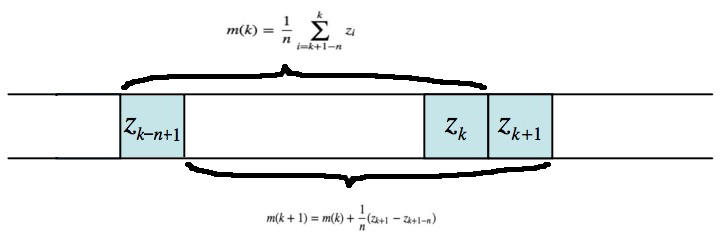Figure 70: “移动平均”原理（一个新的灰度值加入时，同时剔出一个旧的灰度值，无需每次都重新将逐个数值加起来求平均）

$m_{xy} = m(k+1) = \frac{1}{n} \sum_{i=k+2-n}^{k+1} z_i = m(k) + \frac{1}{n}(z_{k+1} - z_{k+1-n})$

71 是“移动平均”可变阈值对手写文本图像进行处理的实例（ $$n$$ 的经验值是平均笔画宽度的5倍，在这里平均笔画宽度是4像素，所以 $$n$$ 取20，而 $$b$$ 可取0.5）。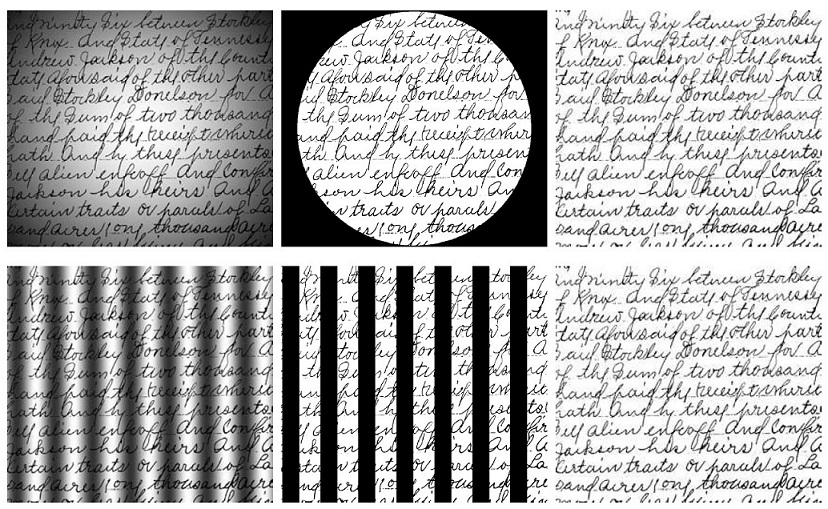Figure 71: 每一排的左图是原图，中图是Otsu方法处理的结果，右图是用“移动平均”进行可变阈值处理的结果

### 4.3 使用形态学分水岭(Watershed)的分割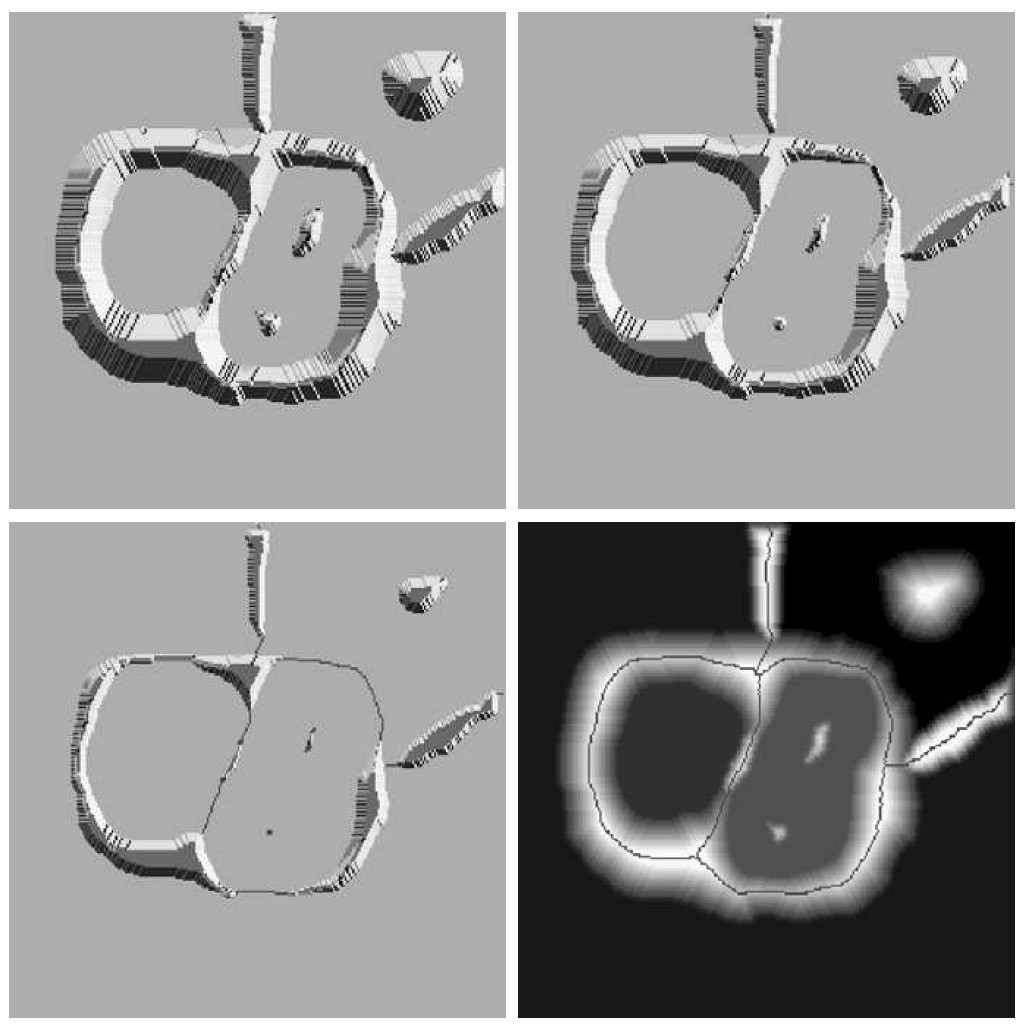Figure 72: 分水岭算法原理：当水从低到高淹没整个地形时，修建水坝来阻止不同的集水盆地中的水聚集

#### 4.3.1 标记的使用(The Use of Markers)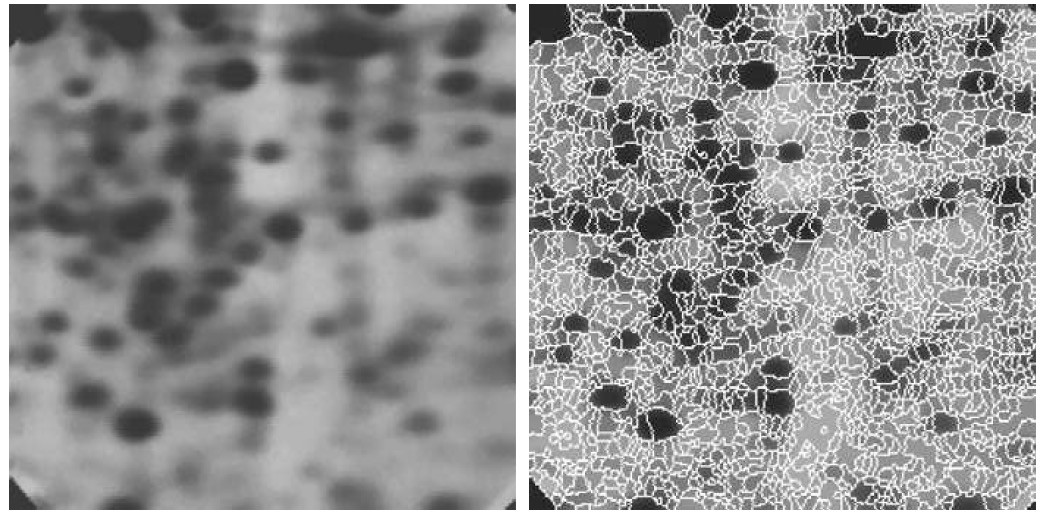Figure 73: 分水岭算法过度分割实例：左图为原图，右图为分水岭算法结果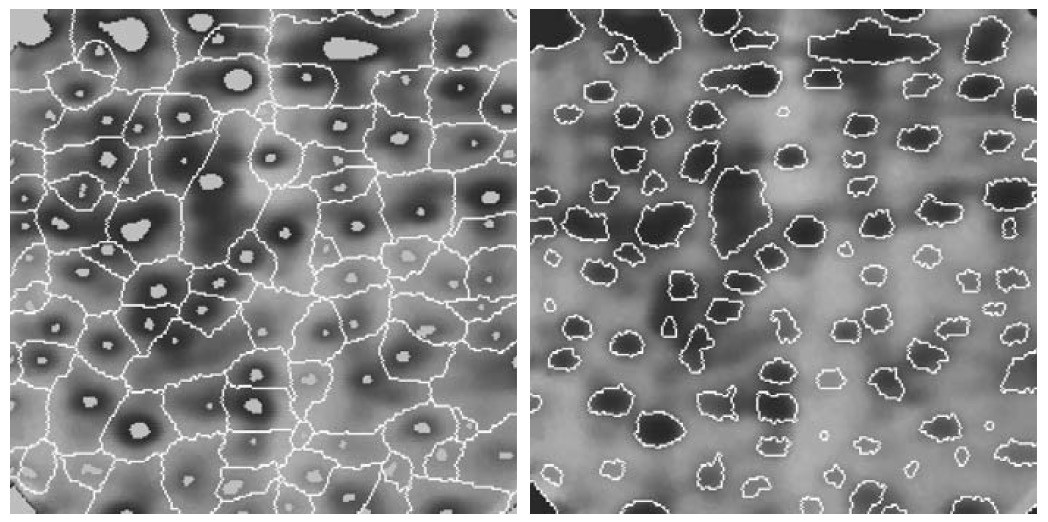Figure 74: 用标记控制过度分割的实例：左图是增加了内部标记（浅灰度区域）和外部标记（分水线）的原图，右图是对应分水岭算法结果

Created: <2015-04-26 Sun 00:00>

Last updated: <2017-12-31 Sun 21:02>

Creator: Emacs 25.3.1 (Org mode 9.1.4)